# Reflection of Light

## Exercise 7(A)

#### Question 1

What do you mean by reflection of light?

The return of light into the same medium after striking a surface is called reflection of light.

#### Question 2

State which surface of the plane mirror reflects most of the light incident on it — the front smooth surface or the back silvered surface.

The back silvered surface of the plane mirror reflects most of the light incident on it.

#### Question 3

Explain the following terms —

(a) plane mirror

(b) incident ray

(c) reflected ray

(d) angle of incidence

(e) angle of reflection.

Draw diagram/diagrams to show them.

(a) Plane mirror — It is made from few mm thick glass plate. One surface of the glass plate is polished to a high degree of smoothness. This forms the front surface of the mirror and the other surface is silvered (i.e., silver mercury or some suitable material is deposited over it). The silvered surface is further coated with some opaque material so as to protect the silvering on it.

The two surfaces of the plane mirror are shown below —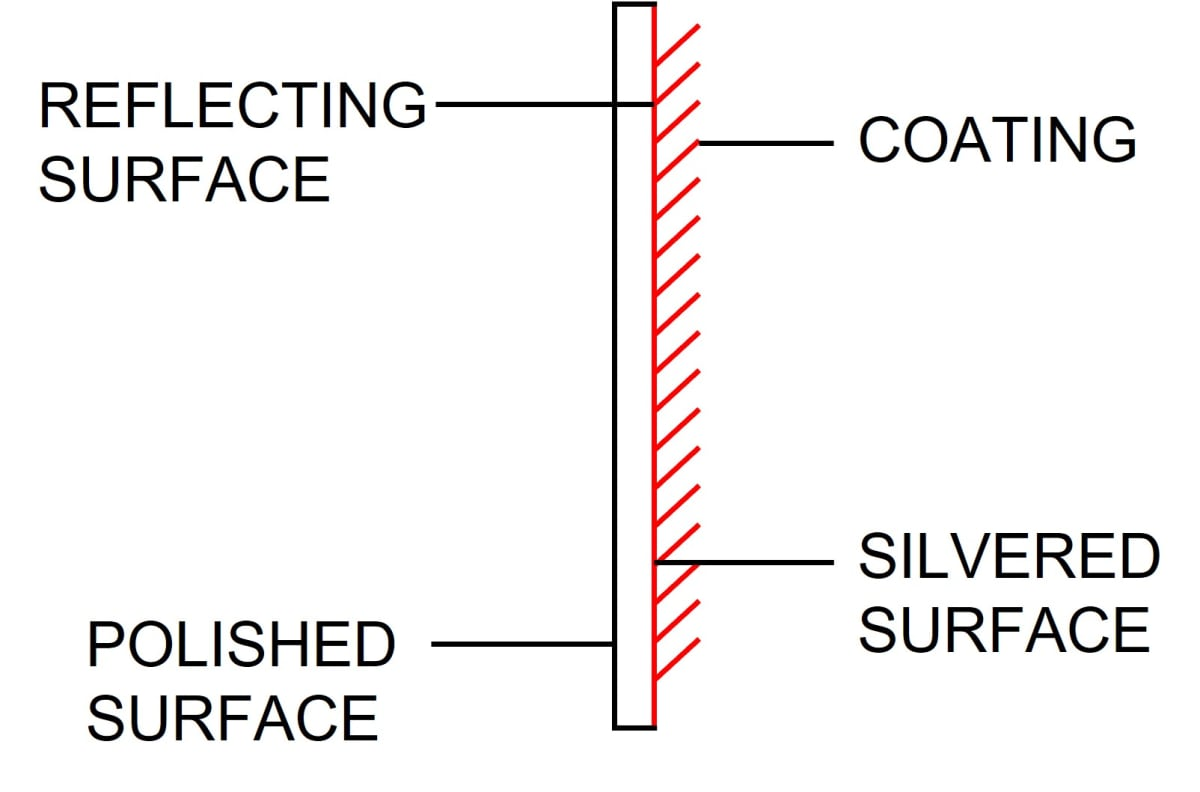(b) Incident ray — the ray of light striking a reflecting surface is called the incident ray.

(c) Reflected ray — the light ray obtained after reflection from the surface in the same medium in which the incident ray is travelling is called reflected ray.

(d) Angle of incidence — The angle which the incident ray makes with the normal at the point of incidence, is called angle of incidence.

It is denoted by the letter i.

(e) Angle of reflection — The angle which the reflected ray makes with the normal at the point of incidence, is called angle of reflection.

It is denoted by the letter r.

Below diagram illustrates the Incident ray, Reflected ray, Angle of incidence and Angle of reflection: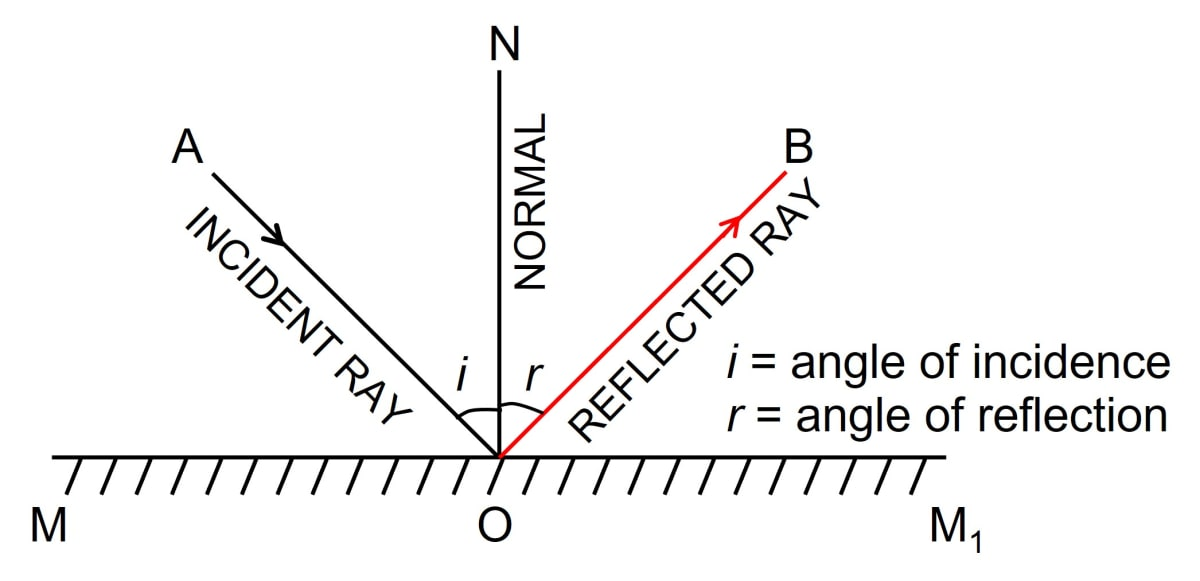#### Question 4

With the help of diagrams, explain the difference between regular and irregular reflection.

Regular reflection — It occurs when a beam of light falls on a smooth and polished surface, such as a plane mirror. In the figure shown below, a parallel beam of light is incident on a plane mirror. The reflected beam is also parallel and it is in a fixed direction. It can be seen only from a particular direction.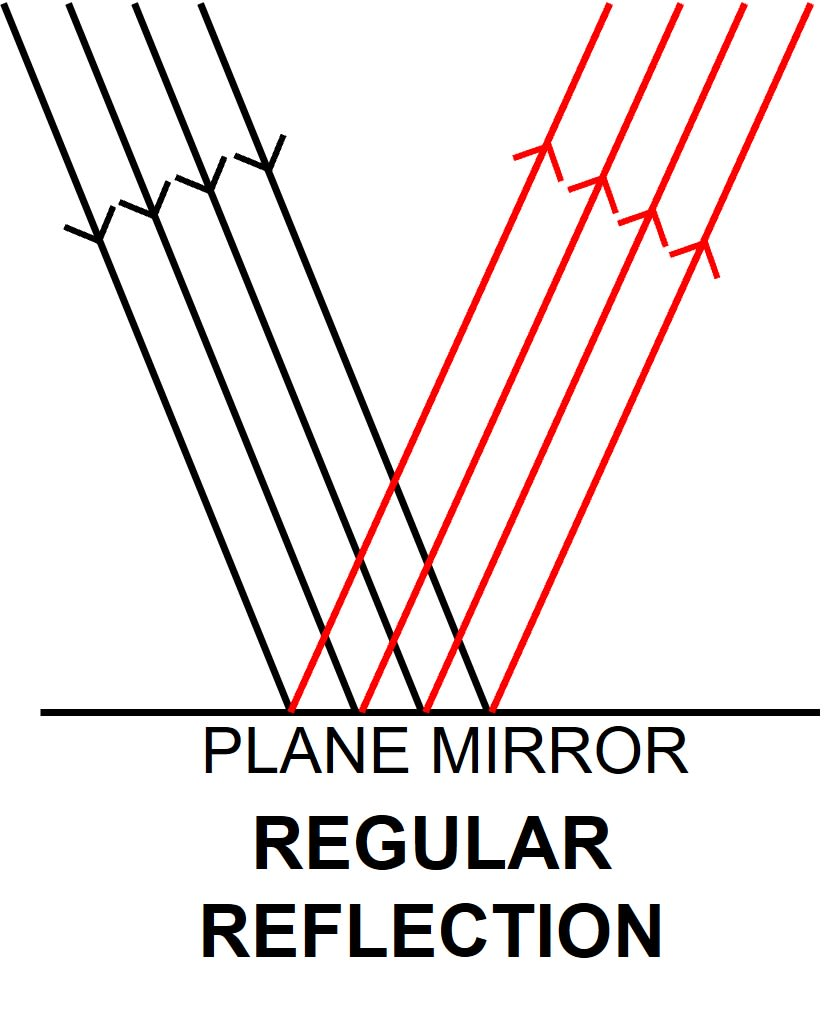Irregular reflection — It occurs when a beam of light falls on a rough or ordinary surface such as the walls of a room or the page of a book which appear smooth but have many small projections over it.

In the figure given below, light rays fall at different points on a rough surface and each ray gets reflected from it obeying the laws of reflection of light. Due to uneven surface at different points, light rays gets reflected in different directions and give rise to diffused or irregular reflection.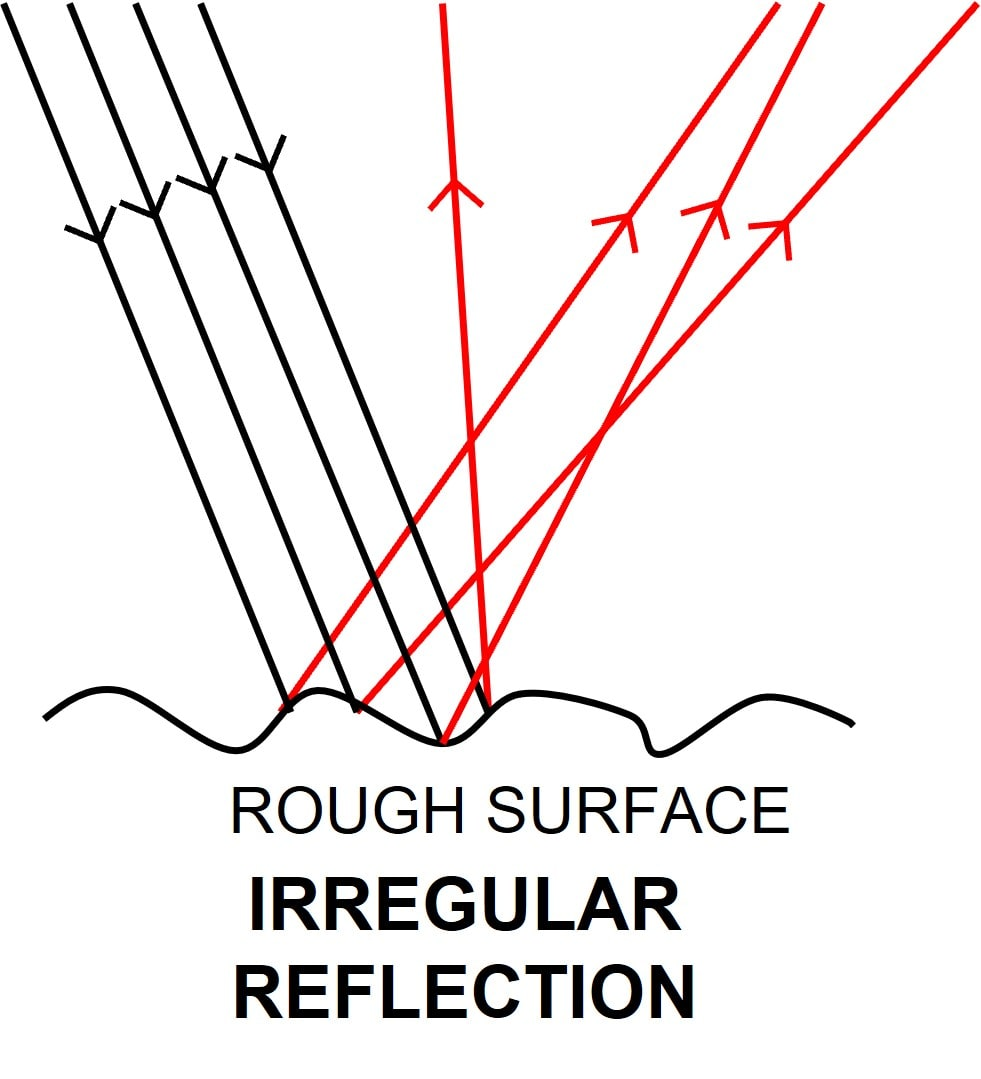As a result, reflected light spreads over a wide area and it does not follow a particular direction. Hence, reflected light can be seen from anywhere.

#### Question 5

Differentiate between reflection of light from a plane mirror and that from a plane sheet of paper.

Reflection of light from a plane mirrorReflection of light from a plane sheet of paper
When a ray of light is incident on a smooth and polished surface such as a plane mirror then regular reflection occurs.When a ray of light is incident of a plane sheet of paper then irregular reflection occurs.
When a parallel beam of light is incident on a plane mirror, the reflected beam is also parallel and it is in a fixed direction .When rays fall at different points on a plane sheet of paper then due to uneven surface at different points, light rays gets reflected in different directions. As a result, The reflected light spreads over a wide area and it does not follow a particular direction.

#### Question 6

State the two laws of reflection of light.

The two laws of reflection are —1. The angle of incidence i is equal to the angle of reflection r (i.e., ∠i = ∠r)
∠AON = ∠BON
2. The incident ray, the reflected ray and the normal at the point of incidence, lie in the same plane.
AO, ON and OB are in one plane.

#### Question 7

State the laws of reflection and describe an experiment to verify them.

The two laws of reflection are —1. The angle of incidence i is equal to the angle of reflection r (i.e., ∠i = ∠r)
∠AON = ∠BON

2. The incident ray, the reflected ray and the normal at the point of incidence, lie in the same plane.
AO, ON and OB are in one plane.

Experimental verification

Fix a sheet of white paper on a drawing board and draw a line MM1 as shown below. On this line, take a point O nearly at the middle of it and draw a line OA such that ∠MOA is less than 90° (say, ∠MOA = 60°). Then draw a normal ON on line MM1 at point O, and place a small plane mirror vertically by means of a stand with it's silvered surface on the line MM1.

Now fix two pins P and Q vertically at some distance (say 5 cm) apart on line OA, on the board. Keeping eye on the other side of the normal (but on the same side of mirror), see clearly the images P'and Q' of the pins P and Q.

Now fix a pin R such that it is in line with the images P' and Q' as observed in the mirror. Now fix one more pin S such that the pin S is also in line with the pin R as well as the images P' and Q' of pins P and Q.

Draw small circles on paper around the positions of pins as in figure. Remove the pins and draw a line OB joining the point O to the pin points S and R.

As we can observe, AO is the incident ray, OB is the reflected ray, ∠AON = i is the angle of incidence and ∠BON = r is the angle of reflection. The angles AON and BON are measured and recorded in the observation table.

The experiment is repeated for the ∠MOA equal to 50°, 40° and 30°.

Observation table —

S. No.Angle of incidence,
i = ∠AON
(in degrees)
Angle of reflection
r = ∠BON
(in degrees)
13030
24040
35050
46060

From the above observation table, we find that in each case, angle of incidence is equal to the angle of reflection. This verifies the first law of reflection.

The experiment is being performed on a flat drawing board, with mirror normal to the plane on which white sheet of paper is being fixed. Since the lower tips of all the four pins lie on the same plane (i.e., the plane of paper), therefore, the incident ray, the reflected ray and the normal at the point of incidence, all lie in one plane. This verifies the second law of reflection.

#### Question 8

A light ray is incident normally on a plane mirror.

(a) What is its angle of incidence?

(b) What is the direction of reflected ray? Show it on a diagram.

(a) When the ray of light is incident normally on a plane mirror the angle of incidence = 0°.

(b) The direction of reflected ray is same as the incident ray i.e., it retraces the path as shown below.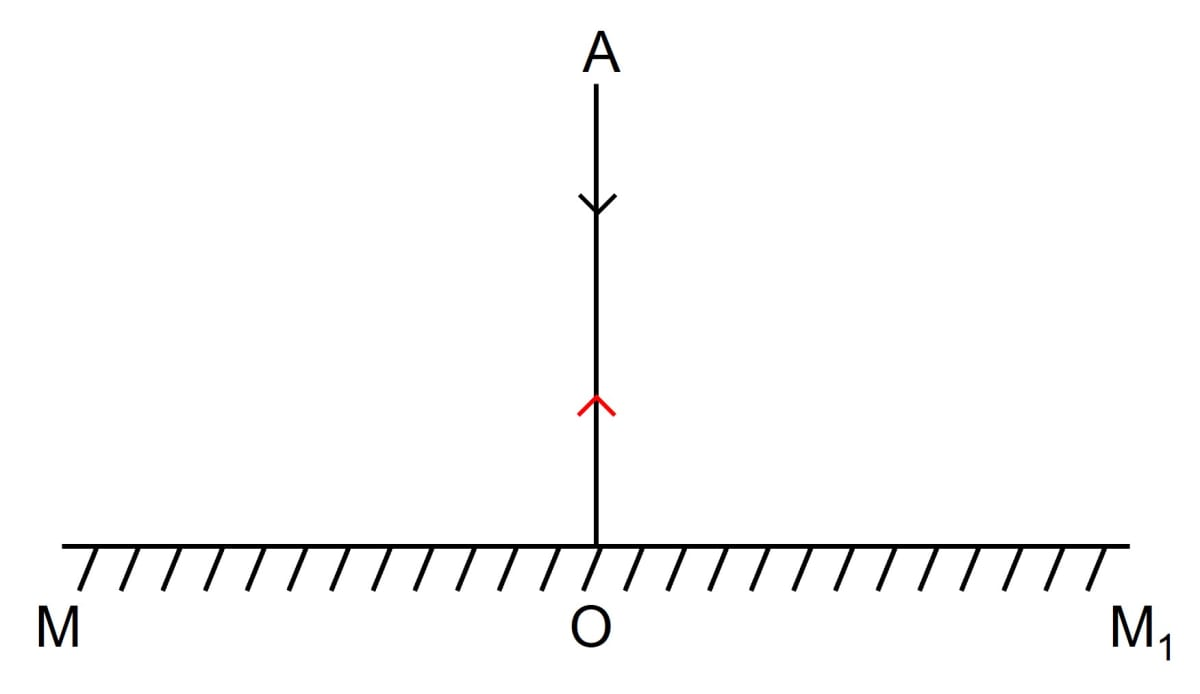#### Question 9

Draw a diagram to show the reflection of a ray of light by a plane mirror. In the diagram, label the incident ray, the reflected ray, the normal, the angle of incidence and the angle of reflection.

Below is the labelled diagram showing the reflection of a ray of light by a plane mirror:#### Question 10

The figure given below shows an incident ray AO and the normal ON on a plane mirror. The angle which the incident ray AO makes with the mirror is 30°.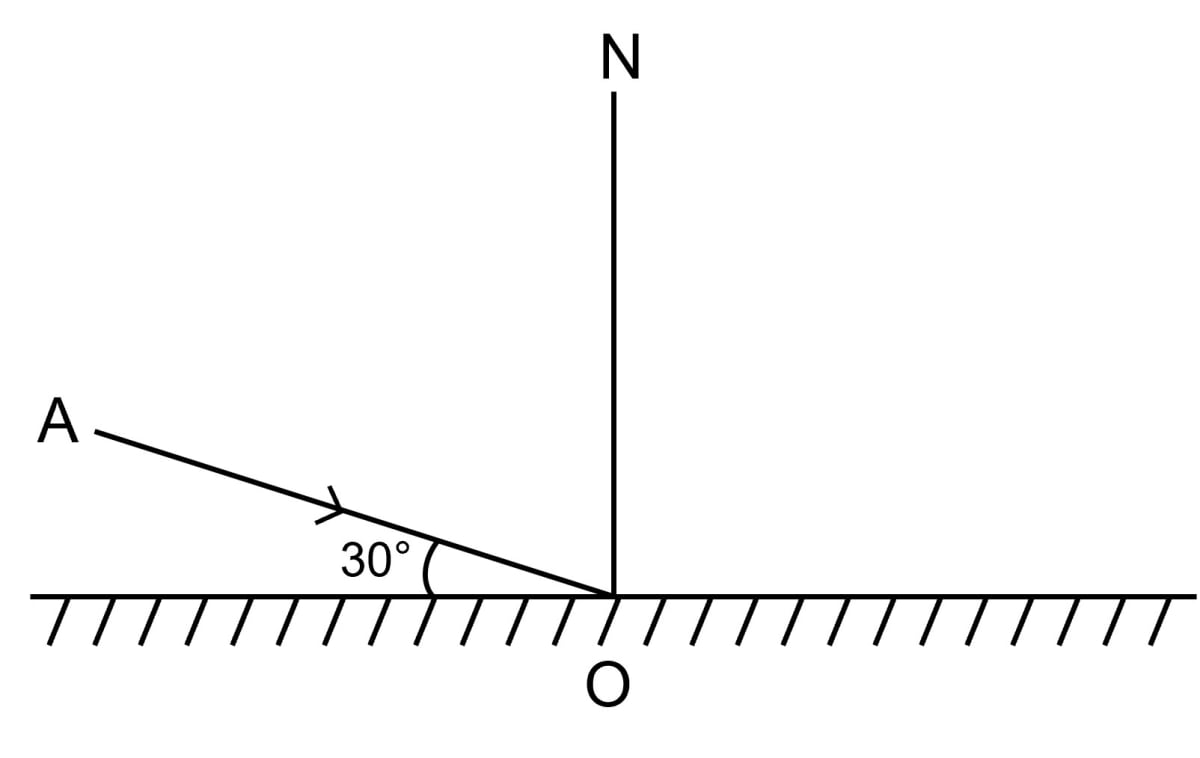(a) Find the angle of incidence.

(b) Draw the reflected ray and then find the angle between the incident and reflected rays.

(a) The angle of incidence = 90° - 30° = 60°

(b) Diagram showing the reflected ray is given below: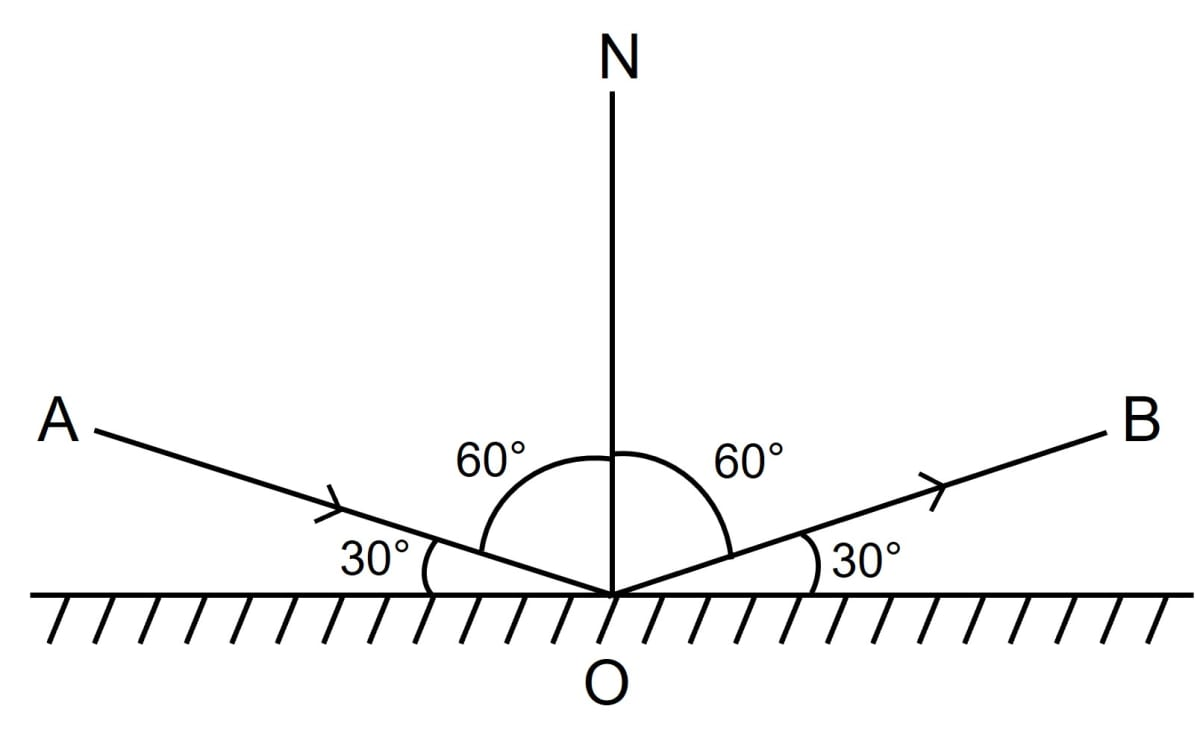The angle between the incident ray and the reflected ray = angle of incidence + angle of reflection

As we know,

Angle of incidence = Angle of reflection = 60°

Therefore, we get,

60° + 60° = 120°

Hence, the angle between the incident ray and reflected ray = 120°

#### Question 11

The diagram below shows a point object P in front of a plane mirror MM1.

(a) Complete the diagram by taking two rays from the point P to show the formation of its image.

(b) In the diagram, mark the position of eye to see the image.

(c) Is the image formed real or virtual? Explain why.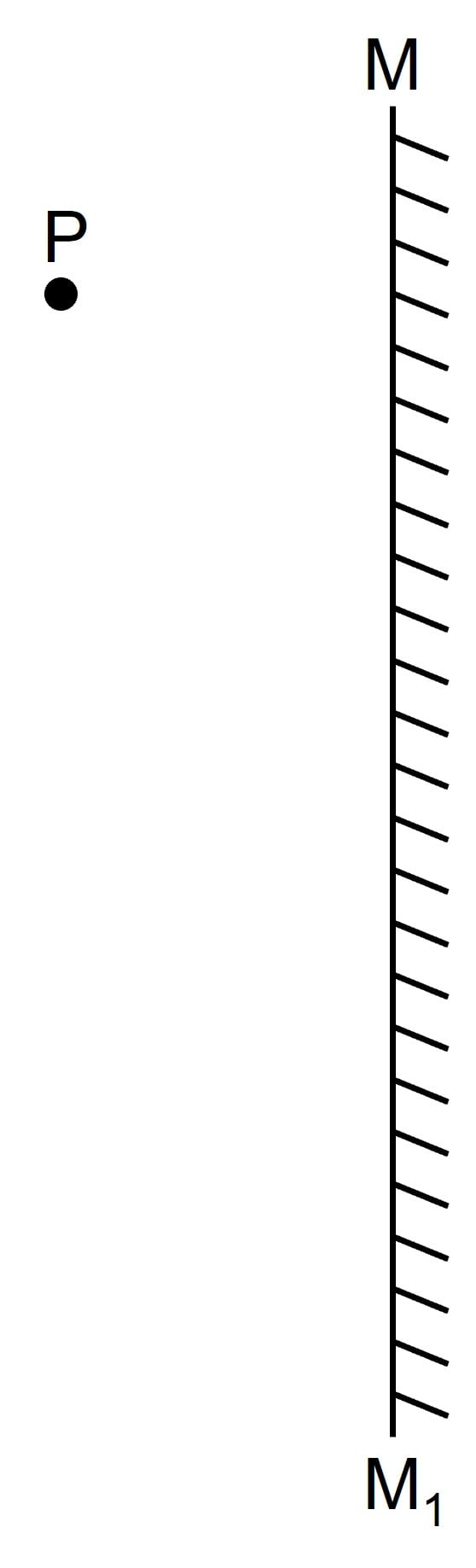Diagram showing the image with the position of the eye marked is given below: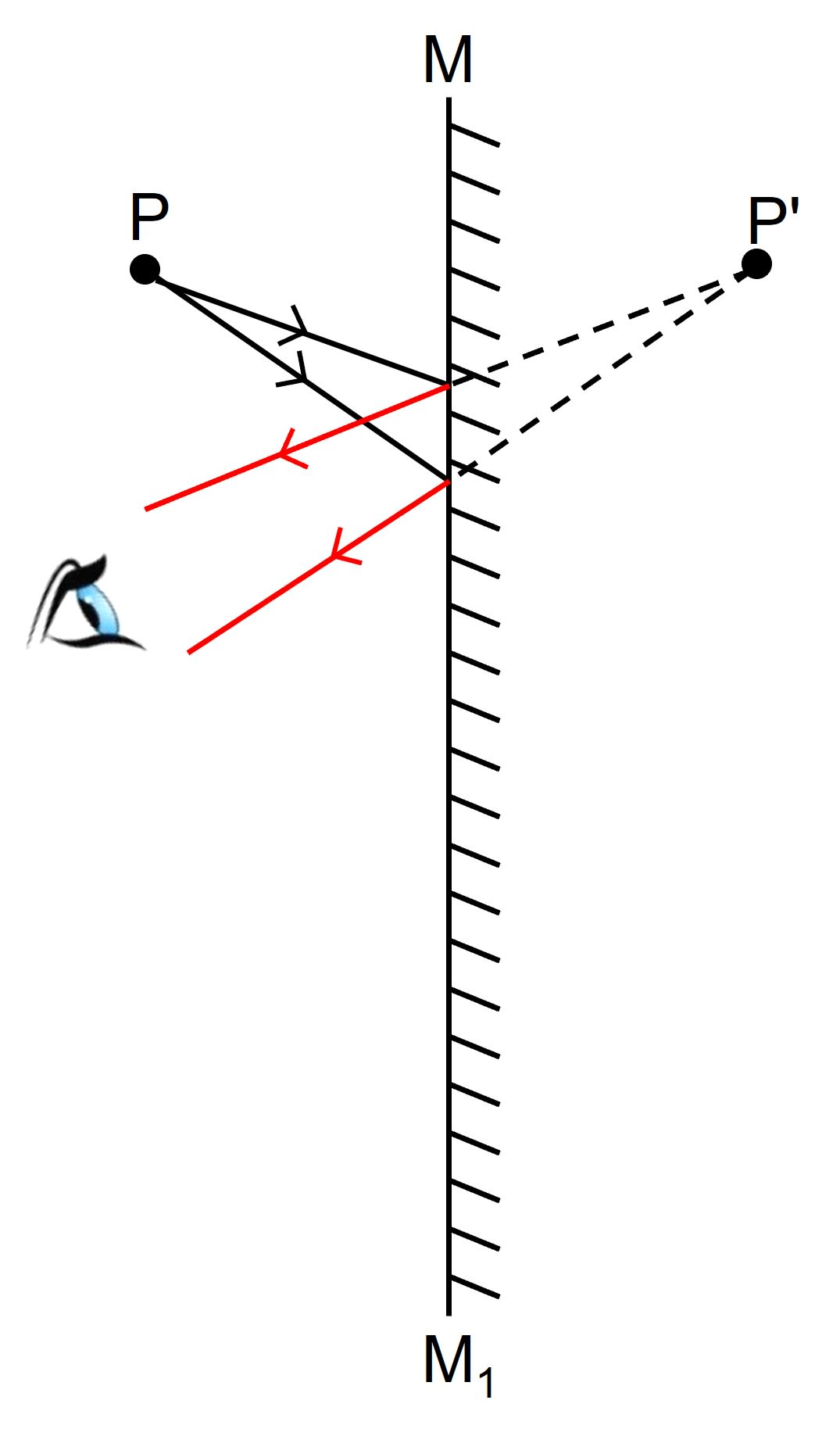The image formed is virtual because the reflected rays appear to meet only when they are produced backwards.

#### Question 12

The diagram shown below shows an object XY in front of a plane mirror MM1. Draw on the diagram, path of two rays from each point X and Y of the object to show the formation of it's image.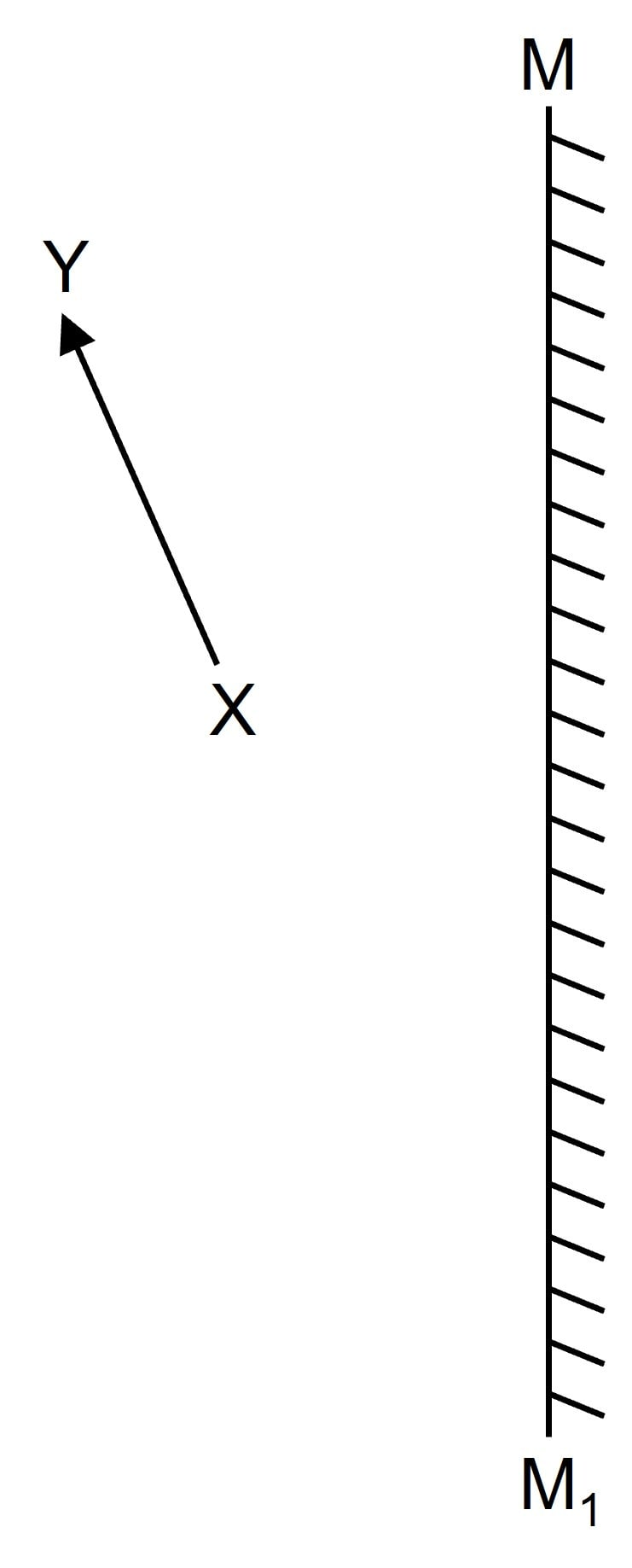Complete diagram showing the formation of the image is given below: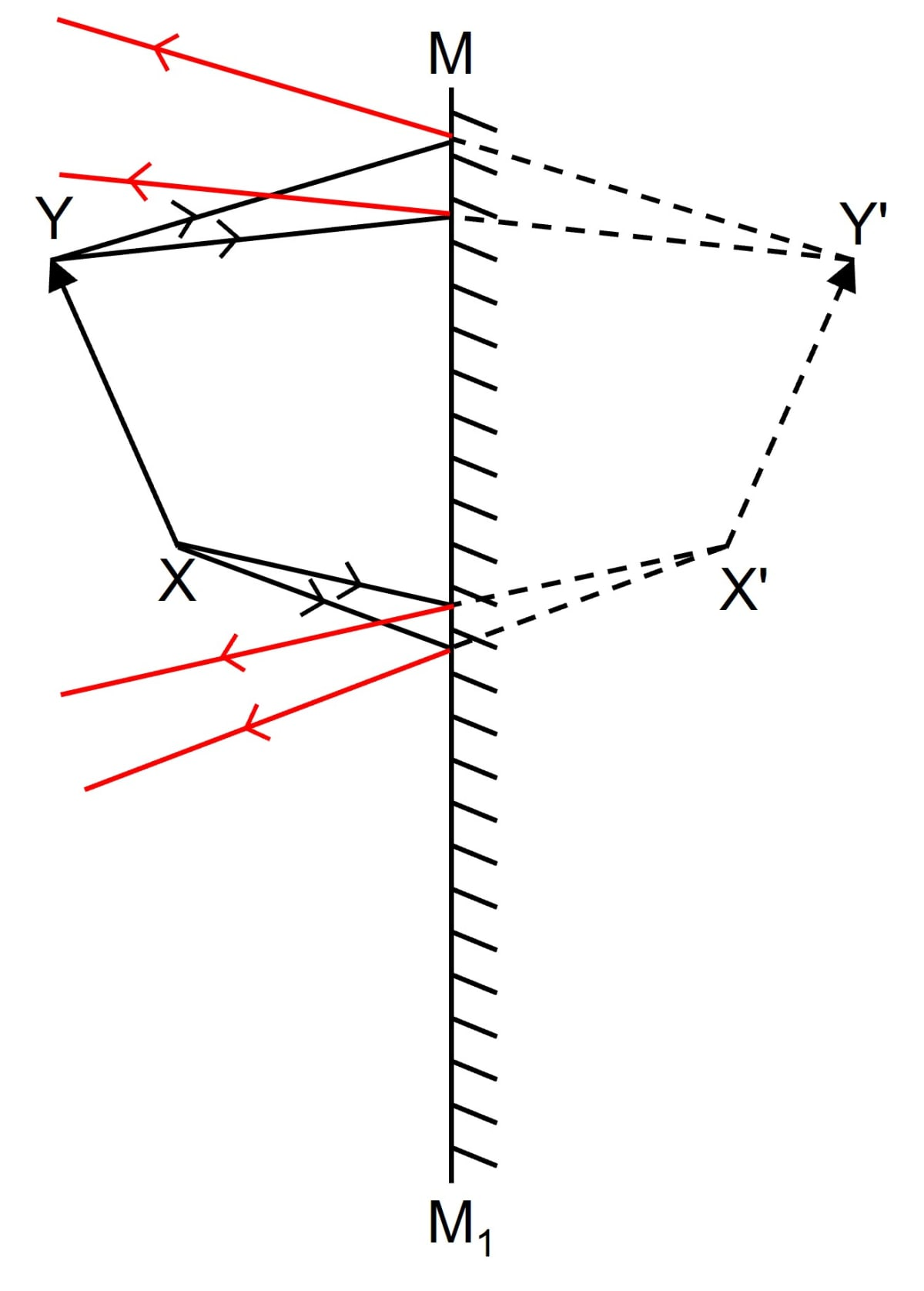#### Question 13

(a) Write three characteristics of image formed by a plane mirror?

(b) How is the position of the image related to the position of the object?

(a) The characteristics of an image formed by a plane mirror are —

1. Image is upright (or erect).
2. Image is virtual.
3. Image is of the same size as the object.

(b) The position of the image is situated at the same perpendicular distance behind the mirror as the object is in front of it.

#### Question 14

Differentiate between a real and a virtual image.

The difference between a real and a virtual image are as follows —

Real imageVirtual image
A real image is formed due to actual intersection of the reflected rays.A virtual image is formed when the reflected rays appear to meet when produced backwards.
A real image can be obtained on a screen.A virtual image cannot be obtained on a screen.
A real image is inverted with respect to the objectA virtual image image is erect with respect to the object.
Example — The image of a distant object formed by a concave mirror.Example — The image of an object formed by a plane mirror or by a convex mirror.

#### Question 15

What is meant by lateral inversion of an image in a plane mirror? Explain it with the help of a ray diagram.

The interchange of the left and right sides in the image of an object in a plane mirror is called lateral inversion.
Below diagram illustrates lateral inversion of an image: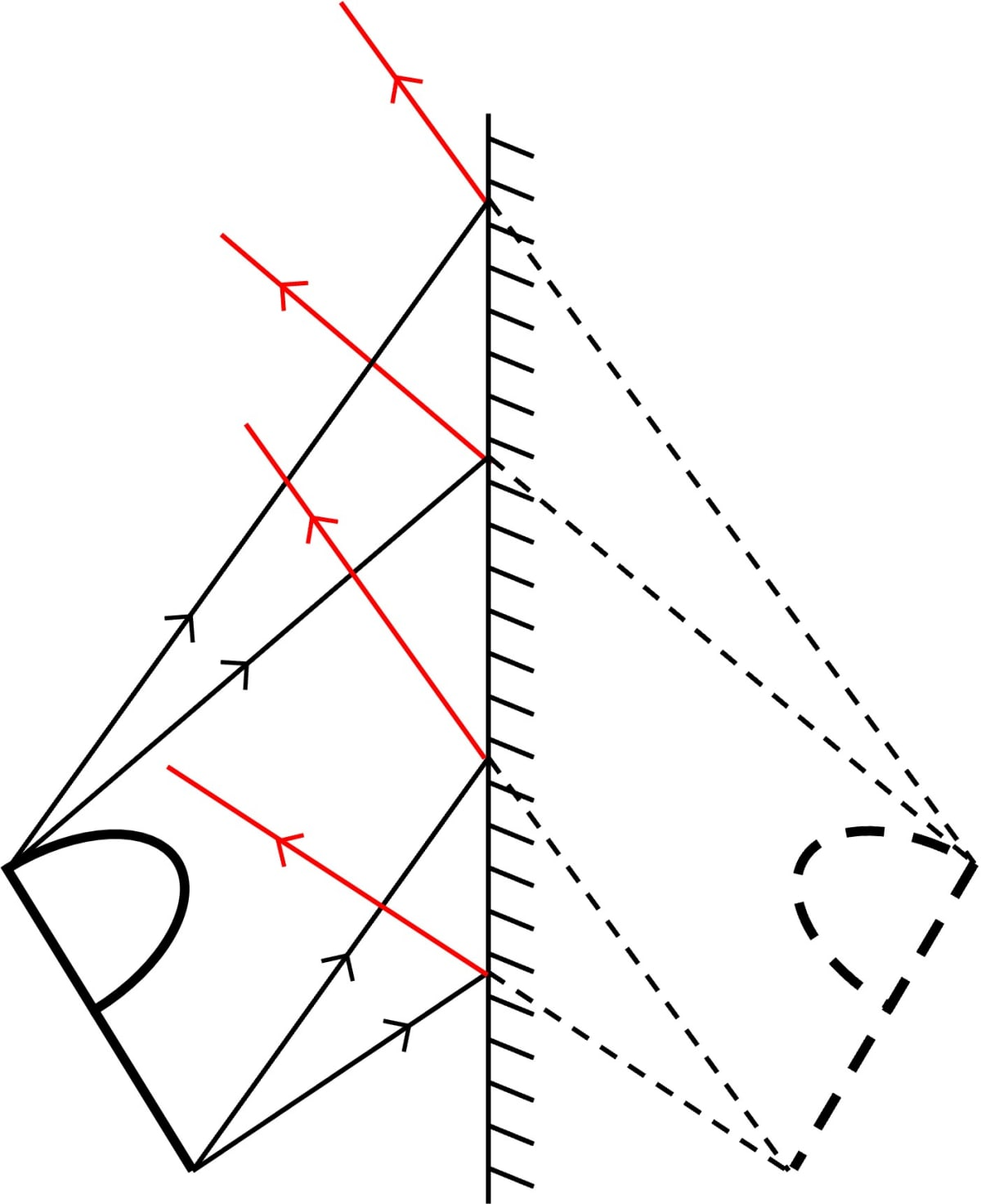#### Question 16

The letters on the front of an ambulance are written laterally inverted like ƎƆИA⅃UꓭMA. Give reason.

The letters on the front of an ambulance are written laterally inverted like ƎƆИA⅃UꓭMA so that the driver of the vehicle moving ahead of the ambulance reads the word laterally inverted as AMBULANCE, in his rear view mirror, and gives way to the ambulance first.

#### Question 17

Why is it difficult to read the image of the text of a page formed due to reflection by a plane mirror?

It is difficult to read the image of the text of a page formed due to reflection by a plane mirror due to lateral inversion.

## Multiple Choice Type

#### Question 1

According to the law of reflection:

1. $\dfrac{i}{r}$ = Constant
2. $\dfrac{\text {sin i}}{\text {sin r}}$ = Constant
3. i + r = Constant
4. i = r ✓

According to the law of reflection the angle of incidence is equal to the angle of reflection.

#### Question 2

The image formed by a plane mirror is:

1. erect and diminished
2. erect and enlarged
3. inverted and of same size
4. erect and of same size ✓

The characteristics of an image formed by a plane mirror are — upright (or erect), virtual and of the same size as the object.

#### Question 3

The image formed by a plane mirror is:

1. real
2. virtual
3. virtual with lateral inversion ✓
4. real with lateral inversion

The characteristics of an image formed by a plane mirror are — upright (or erect), virtual and laterally inverted.

## Numericals

#### Question 1

A ray is incident on a plane mirror. It's reflected ray is perpendicular to the incident ray. Find the angle of incidence.

According to the law of reflection,

angle of incidence = angle of reflection

i.e., i = r

Given,

Reflected ray is perpendicular to incident ray

Hence,

i + r = 90°

Using above law, we get,

i + i = 90°

2i = 90°

Hence,

i = r = 45°

Therefore, angle of incidence = 45°

#### Question 2

A man standing in front of a plane mirror finds his image at a distance 6 metre from himself. What is the distance of a man from the mirror?

Given,

Distance between the man and his image is 6 m

But for a plane mirror,

object distance = image distance

Therefore,

Distance between the man and mirror = the distance between mirror and image

Hence,

the distance of the man and the mirror

$= \dfrac{6}{2} = 3 m$

#### Question 3

An insect is sitting in front of a plane mirror at a distance 1 m from it.

(a) Where is the image of the insect formed?

(b) What is the distance between the insect and it's image?

(a) For a plane mirror, the image is as far behind the mirror as the object is in front of it i.e., the perpendicular distance of image from the mirror is equal to the perpendicular distance of object from the mirror.

Hence, the image of the insect is formed 1 m behind the mirror.

(b) The distance between the insect and it's image = the perpendicular distance of image from the mirror + the perpendicular distance of object from the mirror

= 1 + 1

= 2 m

Hence, the distance between the insect and it's image = 2 m

#### Question 4

An object is kept at 60 cm in front of a plane mirror. If the mirror is now moved 25 cm away from the object, how does the image shift from it's previous position ?

Given,

Initially, the distance of the object A in front of plane mirror M is AM = 60 cm, therefore the image B is at a distance MB = 60 cm from the mirror M behind it. Distance between the object A and it's image B = 60 + 60 = 120 cm

On shifting the mirror by 25 cm away from the object, the new distance of object A from the mirror M' become AM' = 60 + 25 = 85 cm . The new image B' is now at a distance M'B' = 85 cm behind the mirror M'.

Hence, the current distance of the image from the object is 85 cm + 85 cm = 170 cm

Let the reference point be the position of the object,

So, the new distance of the image from the object - the distance of the image from the object initially = distance between the two positions of the image

Hence,

170 cm – 120 cm = 50 cm

Therefore,

the image shifts 50 cm from it's previous position.

#### Question 5

An optician while testing the eyes of a patient keeps a chart of letters 3 m behind the patient and asks him to see the letters on the image of chart formed in a plane mirror kept at distance 2 m in front of him. At what distance is the chart seen by the patient?

Given,

Distance between the man and the mirror = 2 m

Distance between the man and the chart = 3 m

Therefore,

the distance between the chart and the mirror

= distance between the man and the mirror + distance between the man and the chart

= 3 m + 2 m

= 5 m

As the new image is formed on the mirror which is 2 m apart from the man,

So 5 m + 2 m = 7 m

Therefore,

the chart seen by the patient is 7 m away from him

## Exercise 7(B)

#### Question 1

Two plane mirrors are placed making an angle θ in between them. Write an expression for the number of images formed if an object is placed in between the mirrors. State the condition, if any.

When two plane mirrors are placed making an angle θ then number of images formed are:

$n = \dfrac{360\degree}{\theta\degree}$

The conditions are:

1. The number of images are n, when the object is placed asymmetrically between the mirrors and the value of n is odd.
2. The number of images are n - 1, when the object is placed symmetrically (i.e., on the bisector of the angle) between the mirrors and the value of n is even or odd.

#### Question 2

Two plane mirrors are placed making an angle θ° in between them. For an object placed in between the mirrors, if angle is gradually increased from 0° to 180°, how will the number of images change — increase, decrease or remain unchanged?

When two plane mirrors are placed making an angle θ° in between them and an object placed in between the mirrors, and the angle is gradually increased from 0° to 180°, then the number of images decreases.

#### Question 3

How many images are formed for a point object kept in between the two plane mirrors at right angles to each other? Show them by drawing a ray diagram.

Three images will be formed when a point object is placed between two plane mirrors that are at right angles to each other.

The ray diagram is shown below: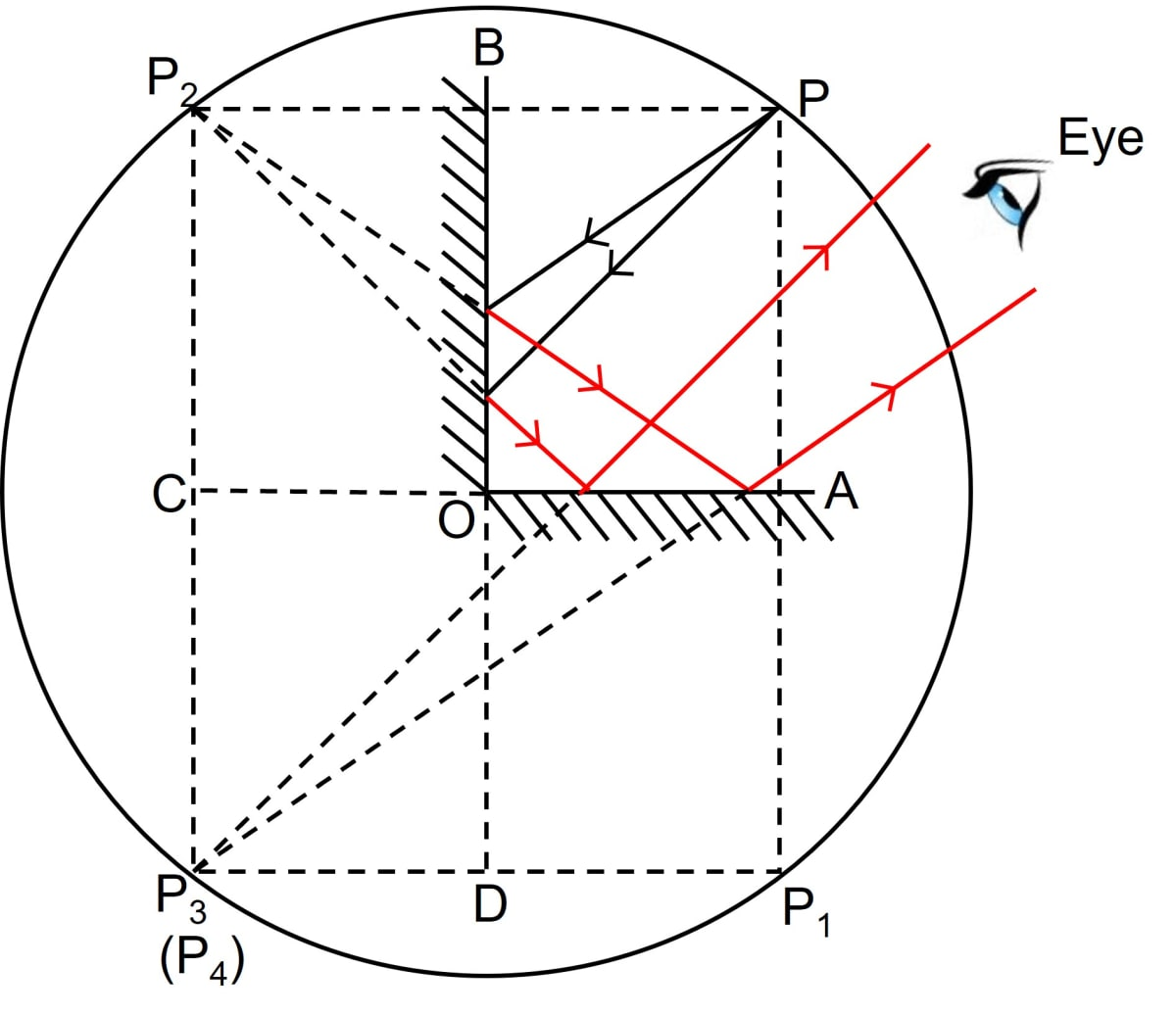#### Question 4

Two plane mirrors are arranged parallel and facing each other at some separation. How many images are formed for a point object kept in between them? Show the formation of images with the help of a ray diagram.

Infinite number of images are formed when two plane mirrors are arranged parallel and facing each other at some separation.

Below ray diagram shows the formation of images: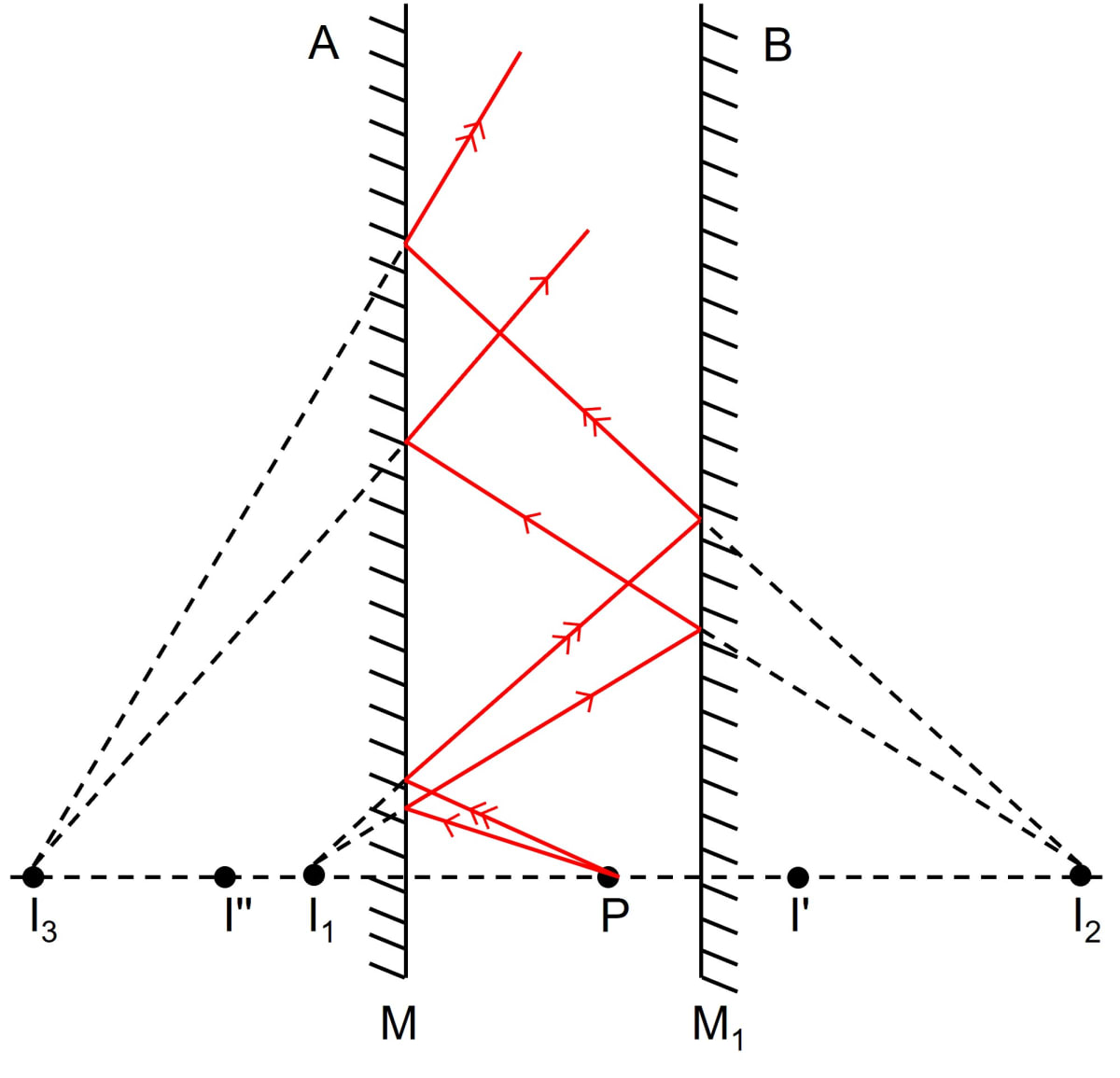#### Question 5

State two uses of a plane mirror.

The uses of a plane mirror are —

(a) The most common and wide use is as a looking glass.

(b) In the optician's room to increase the effective length of the room. It is done by keeping a plane mirror on the front wall and the sign board on the opposite wall, just behind the patient. For the patient the sign board is at nearly double the length of the room.

## Multiple Choice Type

#### Question 1

Two plane mirrors are placed making an angle of 60° in between them. For an object placed in between the mirrors, the number of images formed will be:

1. 3
2. 6
3. 5 ✓
4. infinite

$n = \dfrac{360 °}{\theta°} \\[0.5em] = \dfrac{360°}{60°} = 6$

Hence, number of images formed

= n - 1
= 6 - 1
= 5

Hence, the number of images formed will be 5

#### Question 2

In a barber's shop, two plane mirrors are placed:

1. perpendicular to each other
2. parallel to each other ✓
3. at an angle of 60° between them
4. at an angle of 45° between them.

In a barber's shop, in order to see the hairs at the back of the head two plane mirrors are placed parallel to each other at the front and at the back of the viewer.

## Numericals

#### Question 1

State the number of images of an object placed between the two plane mirrors, formed in each case when the mirrors are inclined to each other at

(a) 90° and

(b) 60°

(a) When two mirrors are kept perpendicular to each other i.e., θ = 90°,

then n = $\dfrac{360°}{90°}$ = 4,

n = 4, is even so number of images is n - 1

Therefore,

n - 1
= 4 - 1
= 3

Hence, for two mirrors kept perpendicular to each other, three images are formed for an object kept in between them.

(b) When two mirrors are kept at 60° to each other,

then n = $\dfrac{360°}{60°}$ = 6

n = 6, is even so number of images is n - 1,

Therefore,

n - 1
= 6 - 1
= 5

Hence, for two mirrors kept at 60° to each other, five images are formed for an object kept in between them.

#### Question 2

An object is placed (i) asymmetrically (ii) symmetrically, between two plane mirrors inclined at an angle of 50°. Find the number of images formed.

(i) When an object is placed asymmetrically between two plane mirrors inclined at an angle of 50° to each other then

$n = \dfrac{360°}{50°} \\[0.5em] = 7.2 \\[0.5em] = 7$

(ii) The number of images formed when the object is placed symmetrically is n-1,

Hence,

7 - 1 = 6.

Therefore, 6 images will be formed when the object is placed symmetrically, between two plane mirrors inclined at an angle of 50°.

## Exercise 7(C)

#### Question 1

What is a spherical mirror?

A reflecting surface which is a part of a sphere is called a spherical mirror.

#### Question 2

Name the two kinds of spherical mirrors and distinguish between them.

Depending on whether the inner or outer surface of the sphere is silvered, spherical mirrors are of two types —

1. Concave mirror
2. Convex mirror

Difference between the two mirrors are —

Concave mirrorConvex mirror
It is made by silvering the outer surface of a part of the hollow sphere, so reflection takes place from the inner surface.It is made by silvering the inner surface of a part of the hollow sphere, so reflection takes place from the bulging surface.
The light rays incident on it converge after reflection.The light rays incident on it diverge after reflection.
The image formed by it is real as well as virtual. For all positions of the object at or beyond the focus, the image is real, while for positions of the object between the focus and the pole, the image is virtual.The image formed by it is always virtual for all positions of the object infront of it.
For object away from the centre of curvature, the image is diminished, for object at the centre of curvature, image is of same size and for object within the centre of curvature, image is diminished.The image is always diminished for all positions of the object infront of it.

#### Question 3

Define the terms pole, principal axis and center of curvature with reference to a spherical mirror.

Pole — The geometric centre of the spherical surface of mirror is called the pole of the mirror.

Principal axis — It is the straight line joining the pole of the mirror to it's centre of curvature.

Centre of curvature — The centre of curvature of a mirror is the centre of sphere of which the mirror is a part.

#### Question 4

Draw suitable diagrams to illustrate the action of (i) concave mirror, and (ii) convex mirror, on a beam of light incident parallel to the principal axis.

(i) Below ray diagram illustrates the action of concave mirror on a beam of light incident parallel to the principal axis: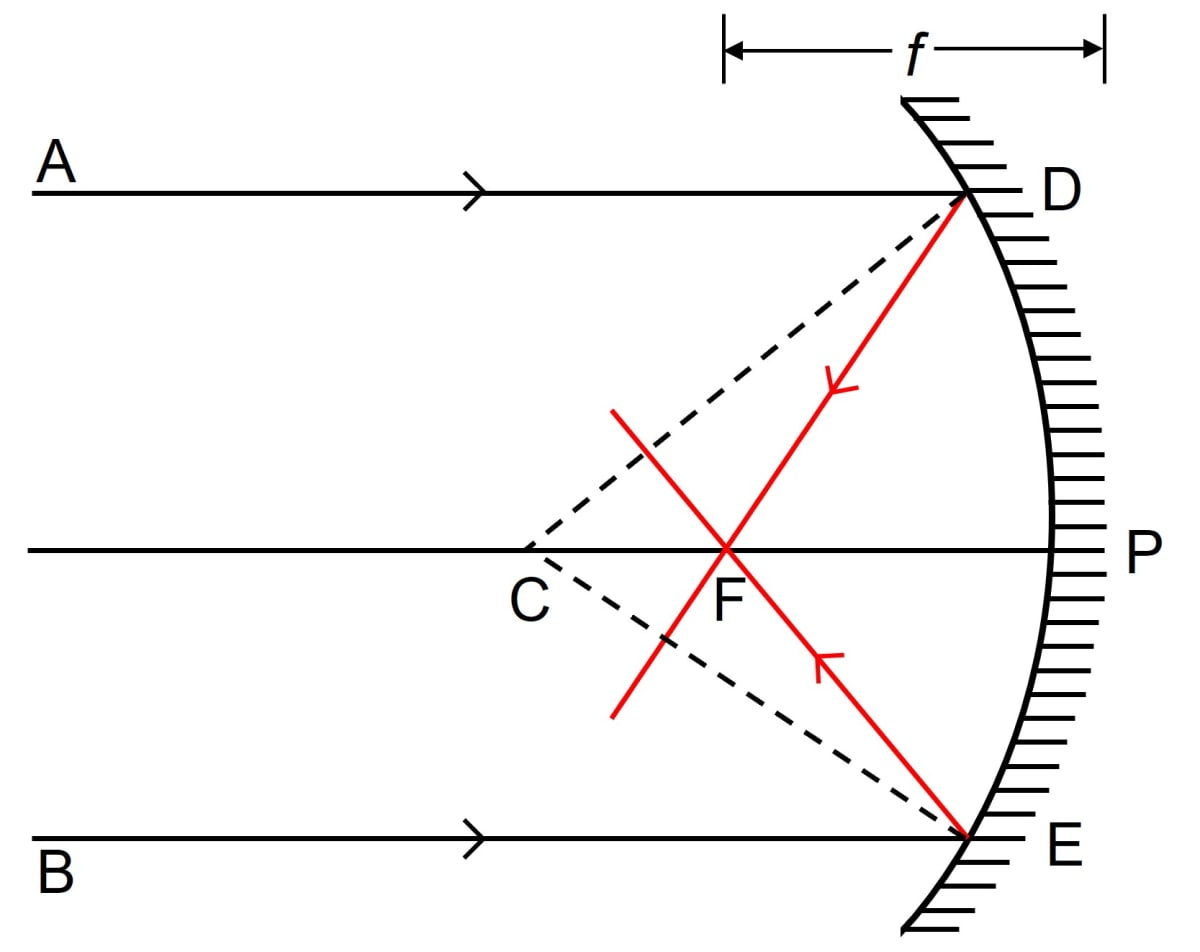(ii) Below ray diagram illustrates the action of convex mirror on a beam of light incident parallel to the principal axis: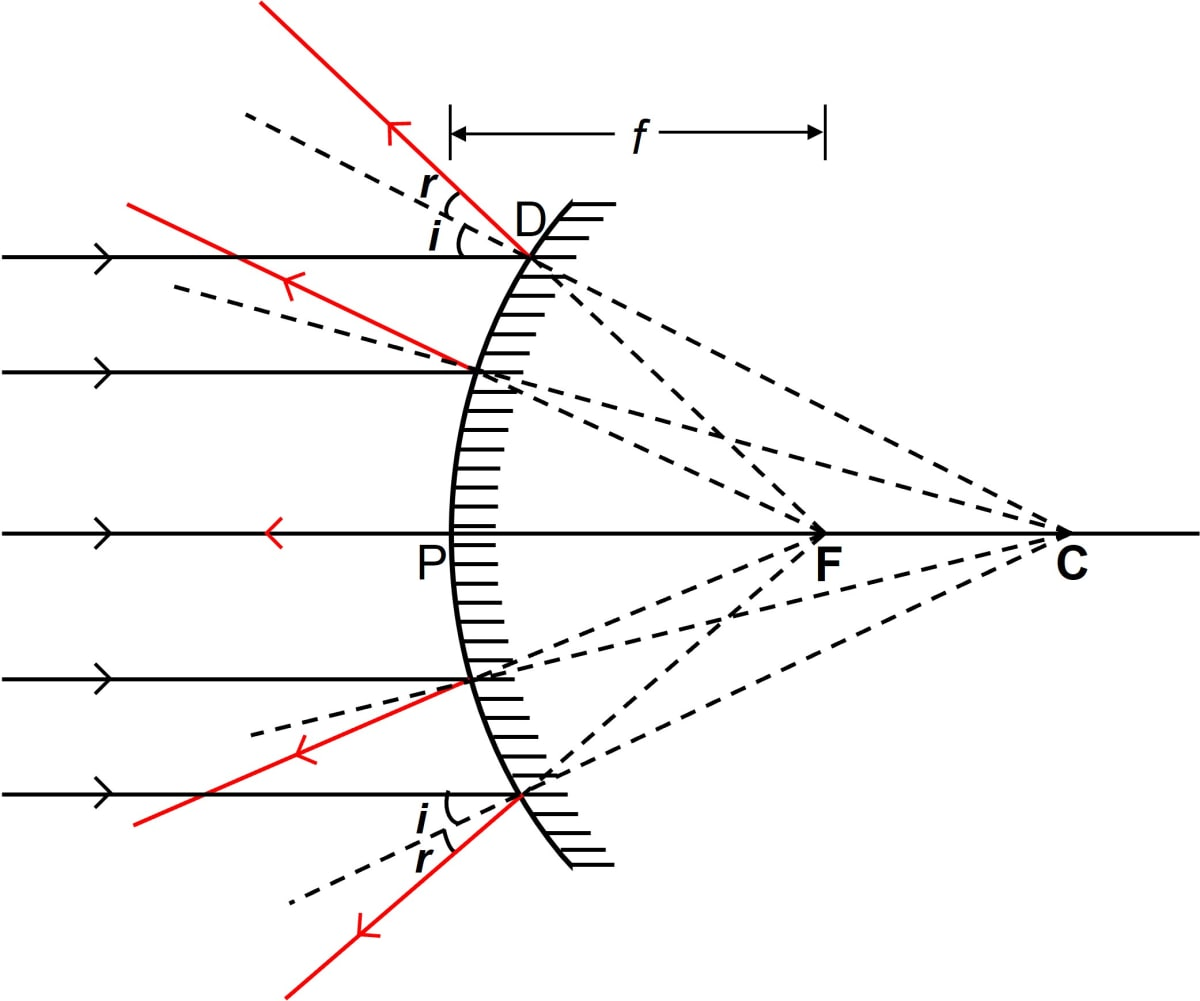#### Question 5

Name the spherical mirror which (i) diverges (ii) converges the beam of light incident on it. Justify your answer by drawing a ray diagram in each case.

(i) A convex mirror diverges a beam of light incident on it.(ii) A concave mirror converges a beam of light incident on it.#### Question 6

Define the terms focus and focal length of a concave mirror. Draw diagram to illustrate your answer.

Focus of a concave mirror — The focus of a concave mirror is a point on the principal axis through which the light rays incident parallel to the principal axis, pass after reflection from the mirror.Focal length of a concave mirror — The distance of focus F from the pole P of the mirror is called the focal length of the mirror.

i.e., focal length f = PF.

#### Question 7

Explain the meaning of the terms focus and focal length in case of a convex mirror, with the help of a suitable ray diagram.

Focus of a convex mirror — The focus of a convex mirror is a point on the principal axis from which, the light rays that are incident parallel to the principal axis, appear to come, after reflection from the mirror.Focal length of a concave mirror — The distance of focus F from the pole P of the mirror is called the focal length of the mirror.

i.e., focal length f = PF.

#### Question 8

State the direction of incident ray which after reflection from a spherical mirror retraces it's path. Give a reason to your answer.

When the incident ray is directed towards the centre of curvature, after reflection from a spherical mirror, it retraces it's path.

It is because the ray is normal to the spherical mirror, so ∠i (angle of incidence) = 0, therefore, ∠r (angle of reflection) = 0.

#### Question 9

(i) Name the mirrors shown in figure (a) and (b).

(ii) In each case (a) and (b), draw the reflected rays for the given incident rays and mark focus by the symbol F.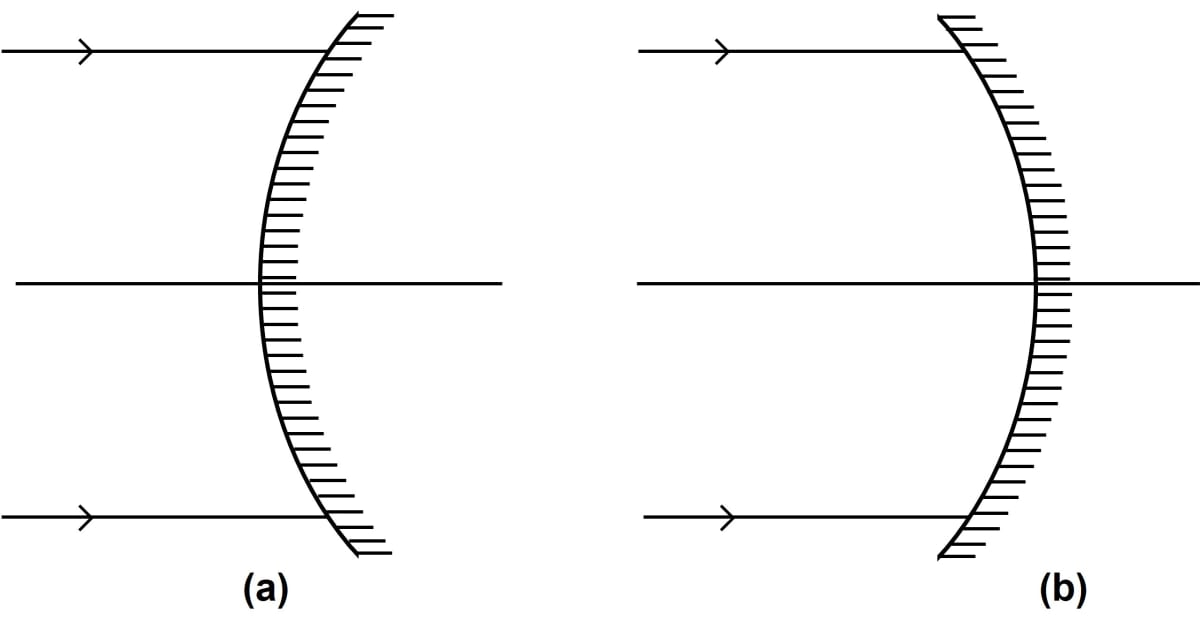(i) The figure (a) shows convex mirror and figure (b) shows concave mirror.

(ii) Diagrams showing reflected rays are shown below:

(a)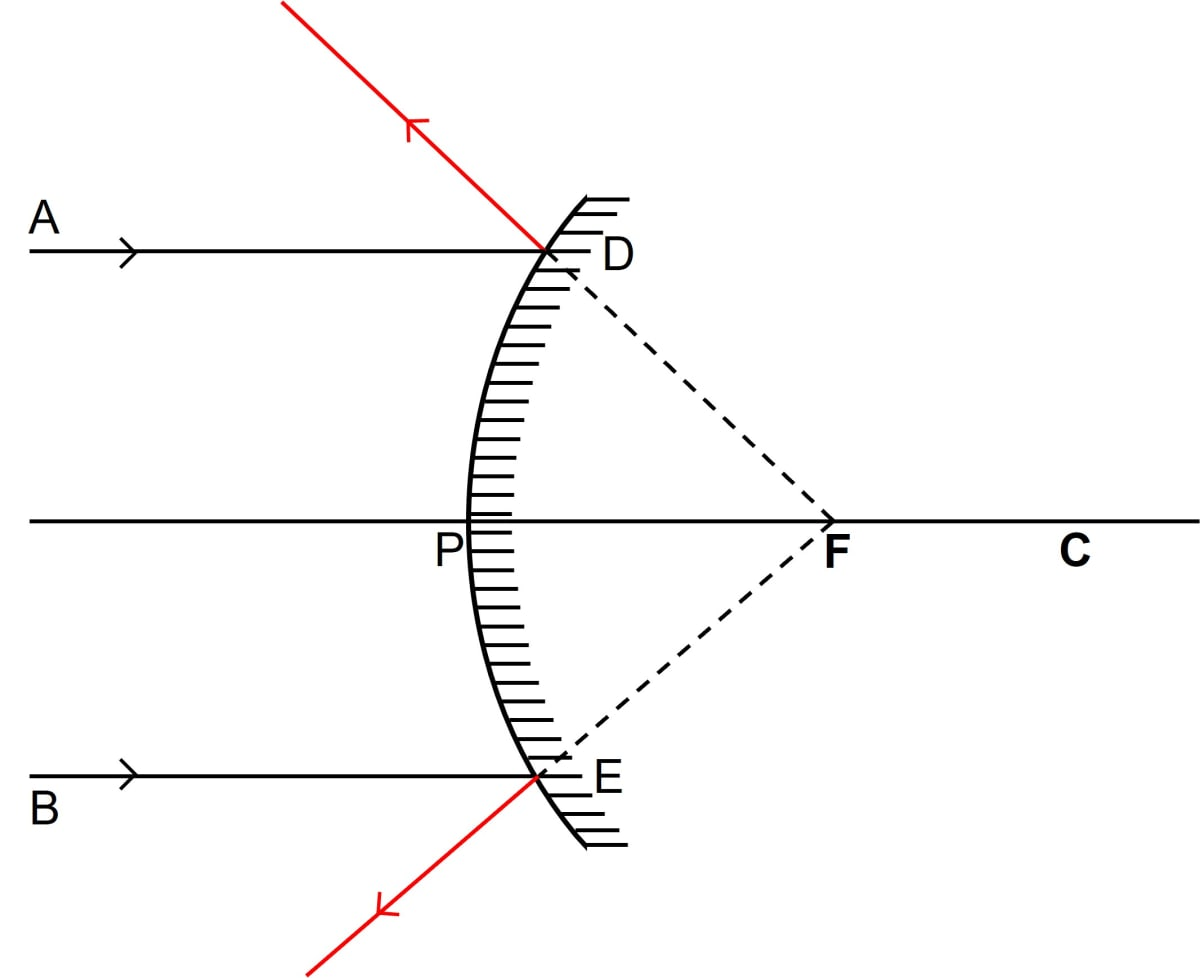(b)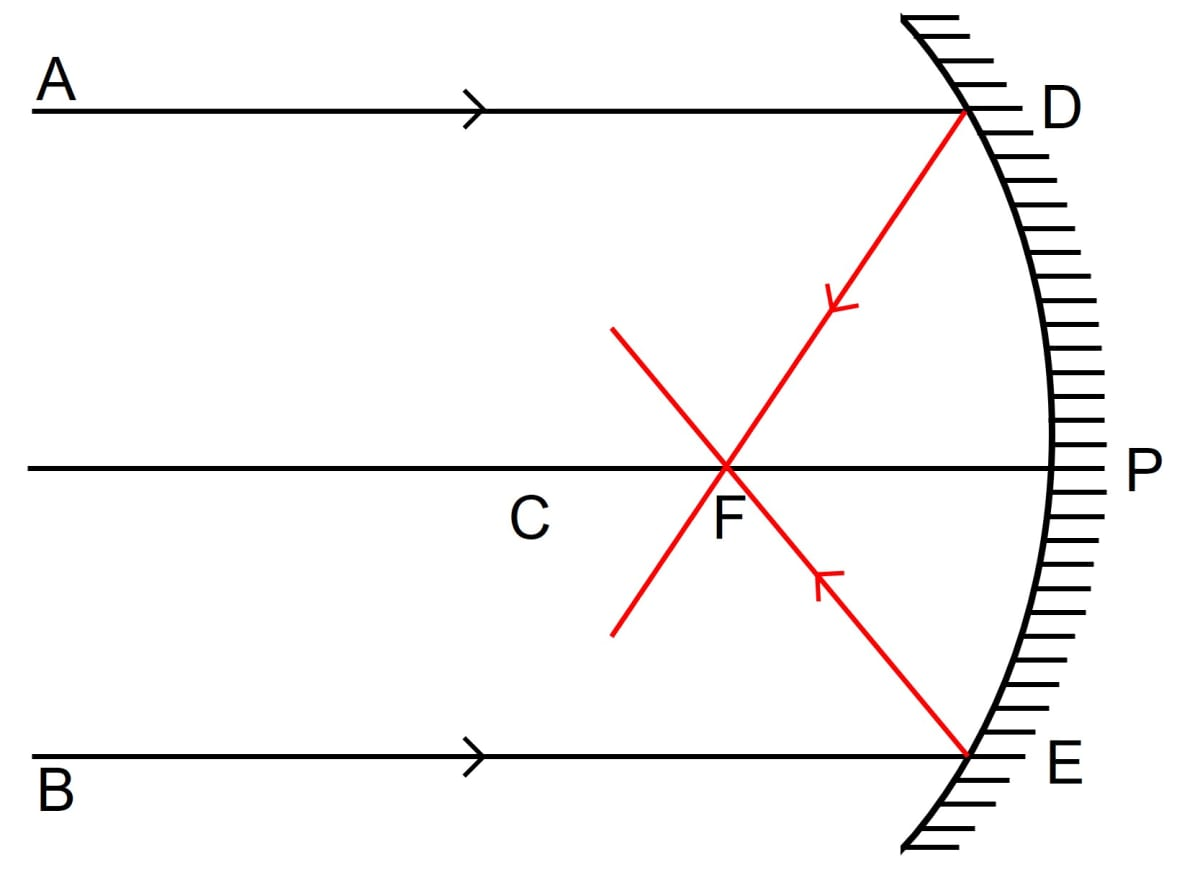#### Question 10

Complete the following diagrams in figure by drawing the reflected rays for the incident rays 1 and 2.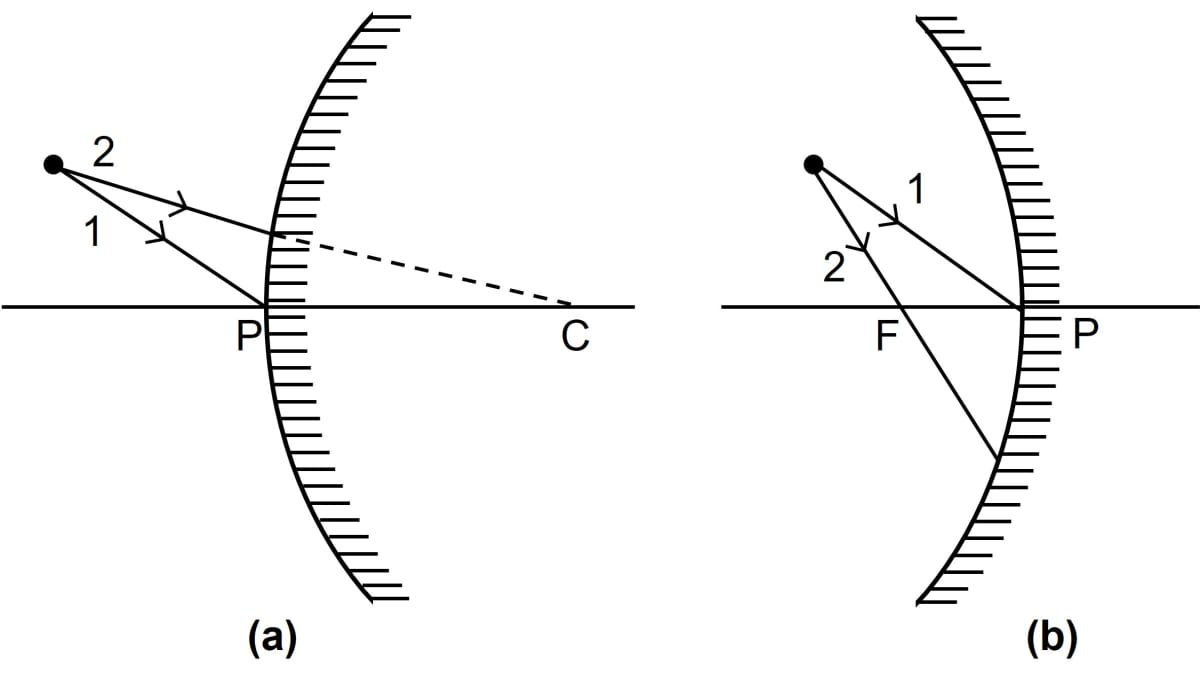Below are the completed diagrams showing the reflected rays for the incident rays 1 and 2:

(a)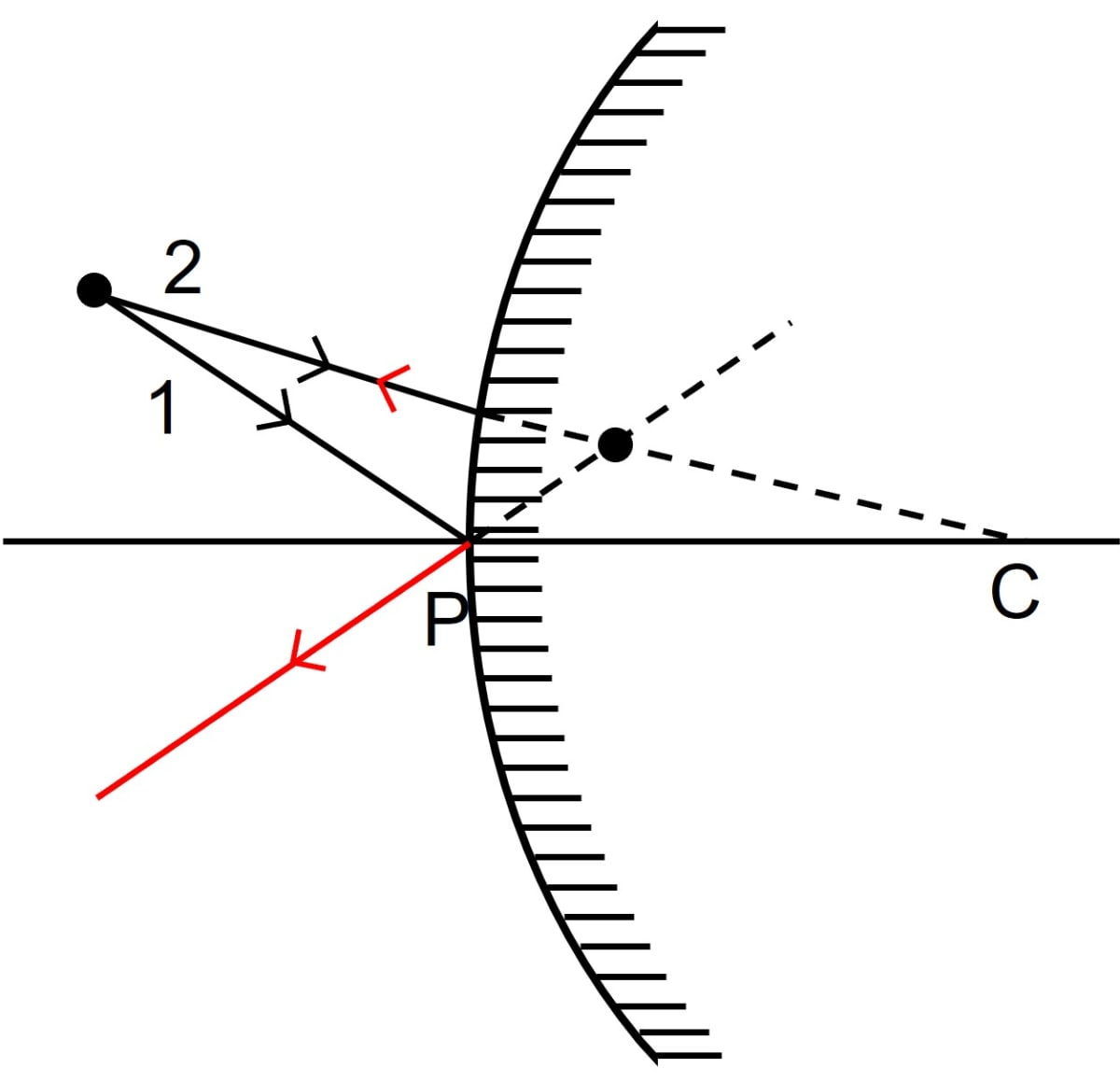(b)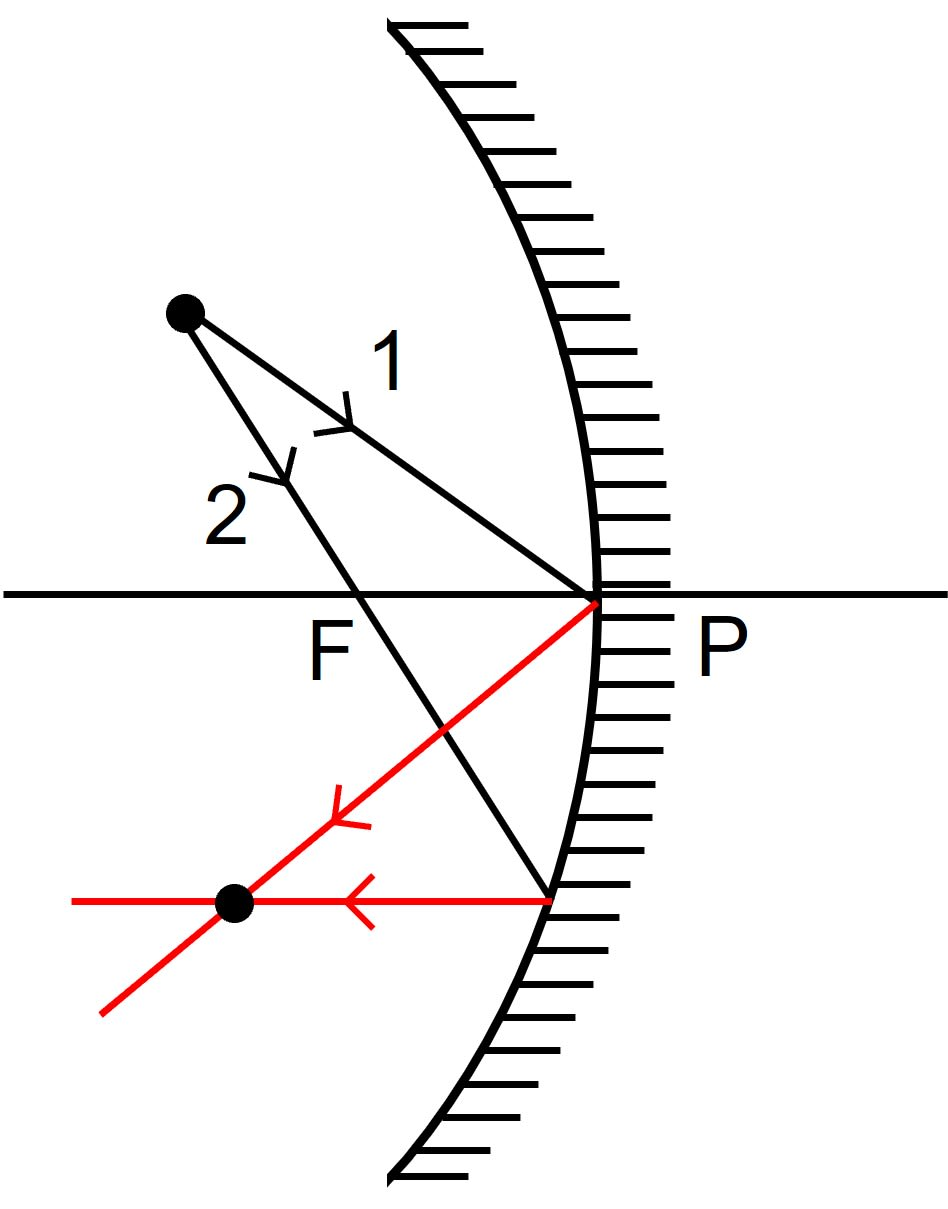#### Question 11

Complete the following diagrams shown in figure by drawing the reflected ray for each of the incident ray A and B.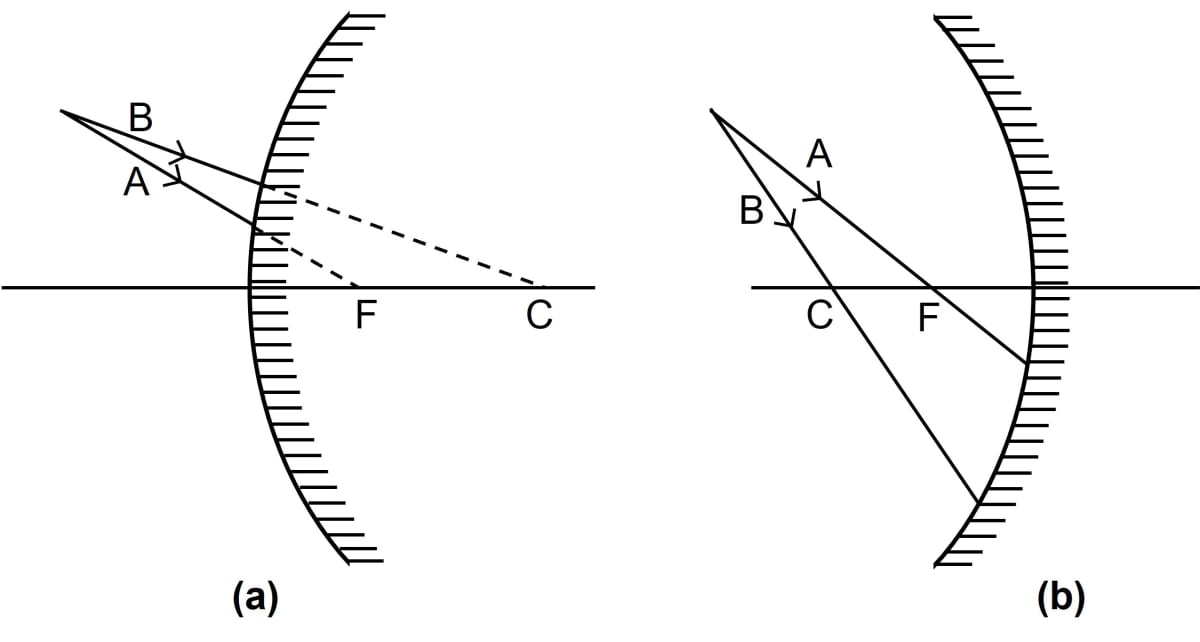Below are the completed diagrams showing the reflected rays for the incident rays A and B:

(a)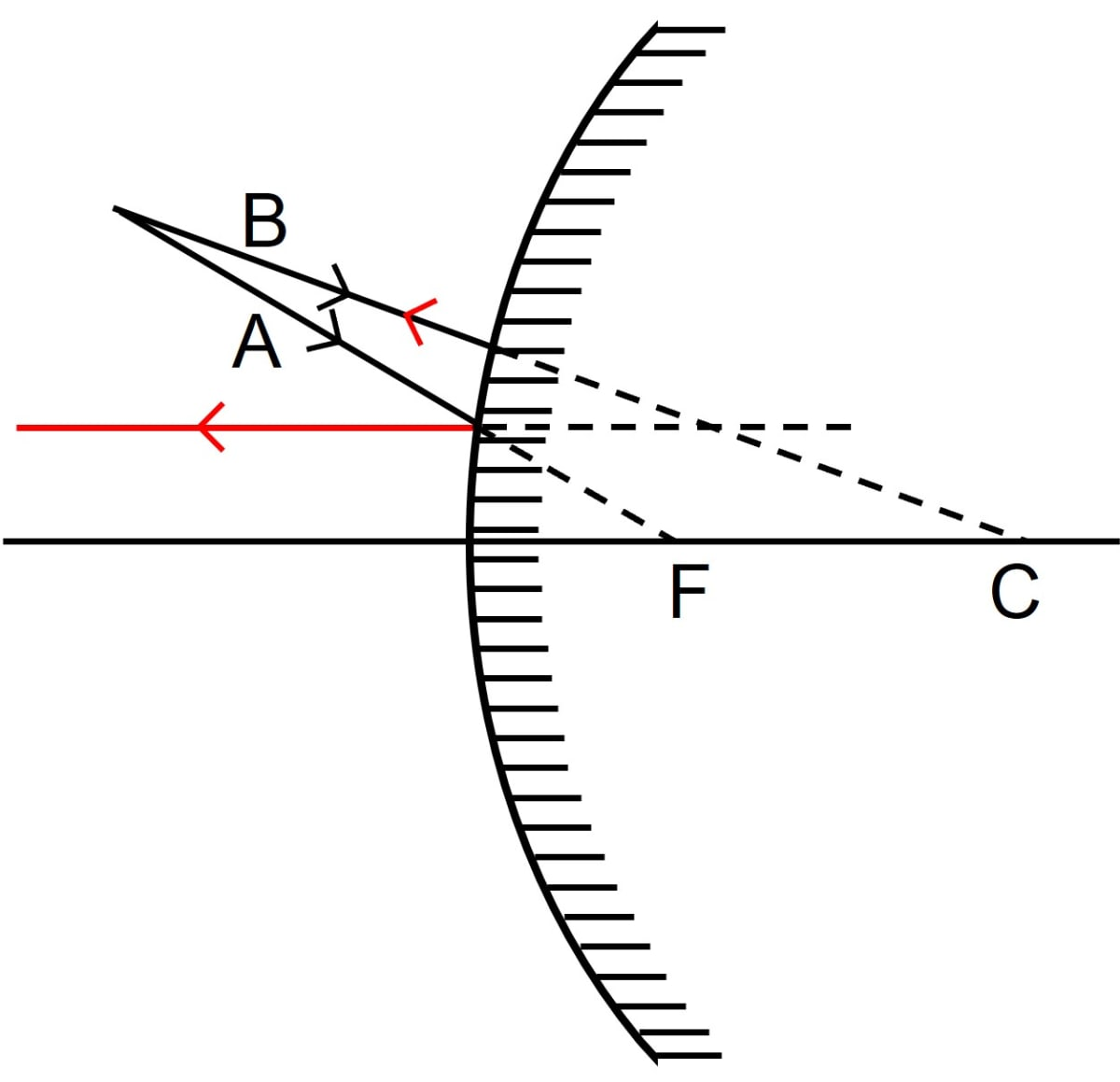(b)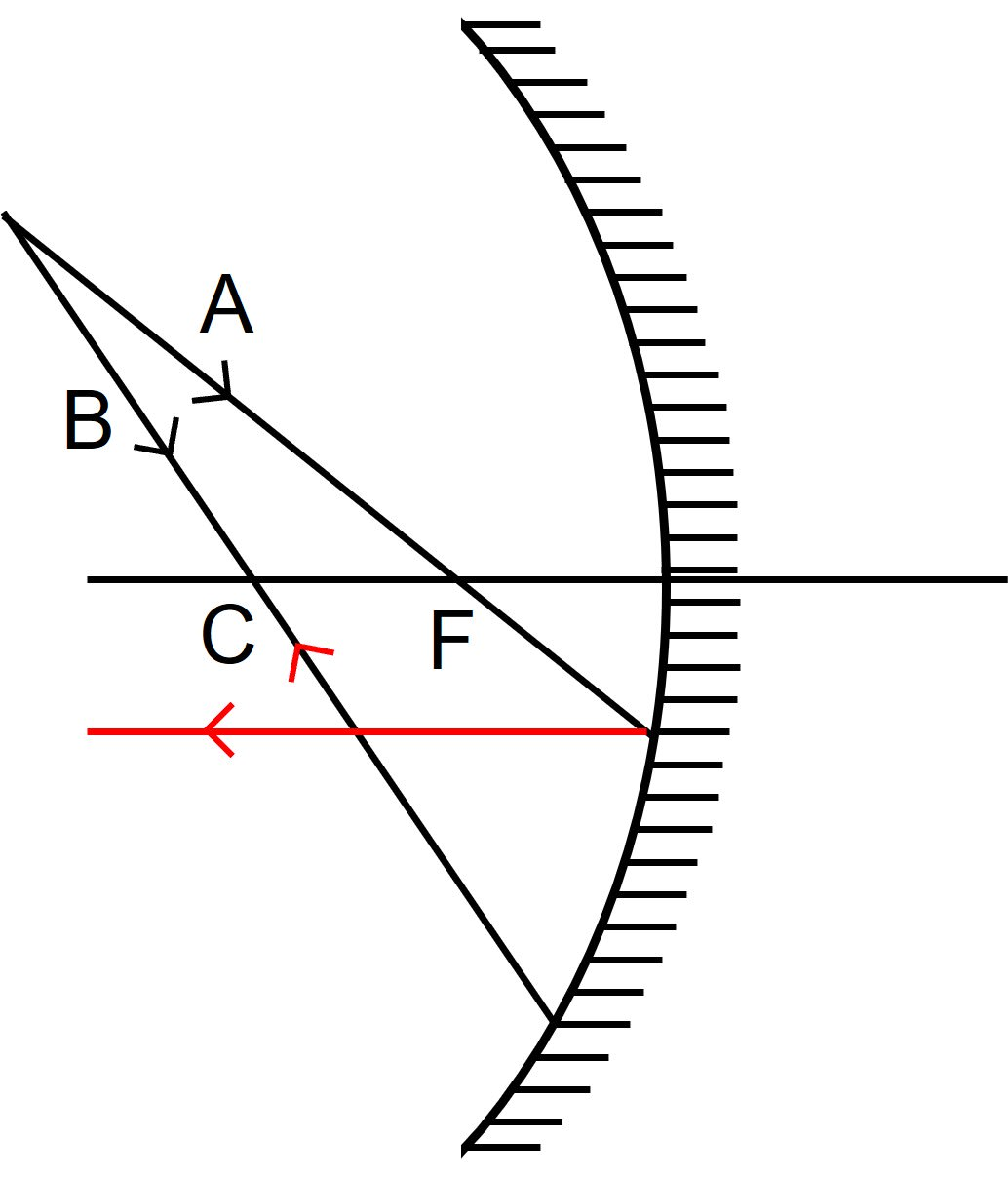#### Question 12

State the two convenient rays that are chosen to construct the image by a spherical mirror for a given object? Explain your answer with the help of suitable ray diagrams.

The two convenient rays, chosen to construct the image by a spherical mirror are —

(i) A ray that passes through the center of curvature.

A line joining the centre of curvature to any point on the surface of mirror is normal to the mirror at that point, therefore a ray AD passing through the center of curvature C (or appearing to pass through through the centre of curvature C) is incident normally on the spherical mirror.

Since it's angle of incidence is zero, therefore the angle of reflection will also be zero and the ray AD gets reflected along it's own path DA as shown below:(ii) A ray parallel to the principal axis.

A ray of light AD incident parallel to the principal axis, after reflection passes either through the focus F(in a concave mirror) or will appear to come from the focus F (in a convex mirror) along DB as shown below: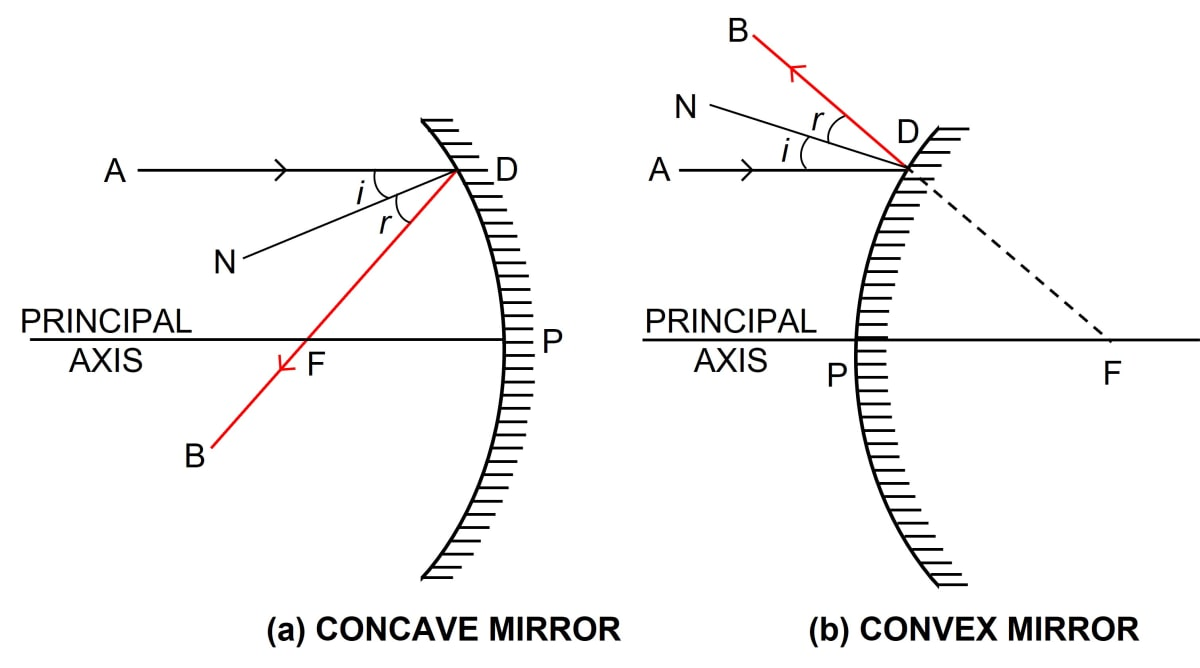#### Question 13

Figure shows a concave mirror with it's pole at P, focus F and center of curvature C. Draw ray diagram to show the formation of image of an object OA.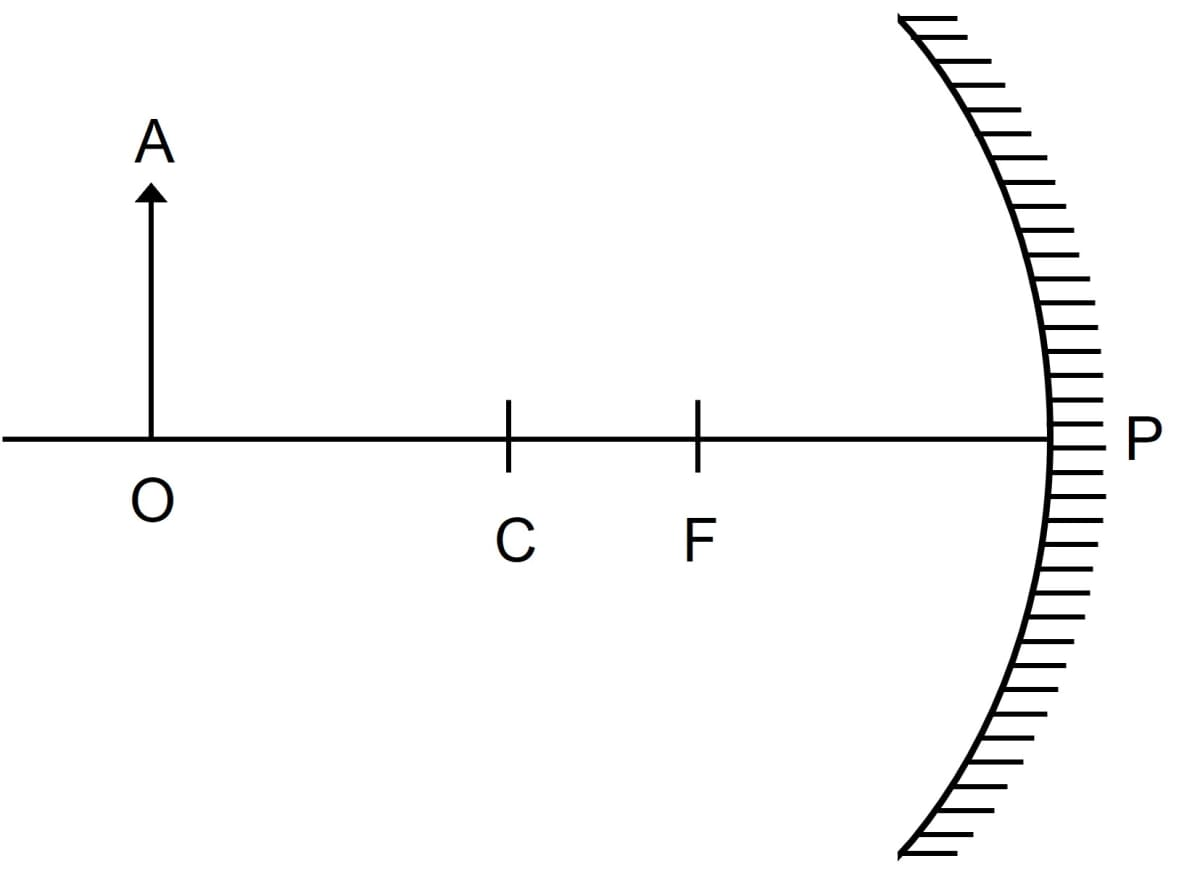Ray diagram showing the formation of the image is given below: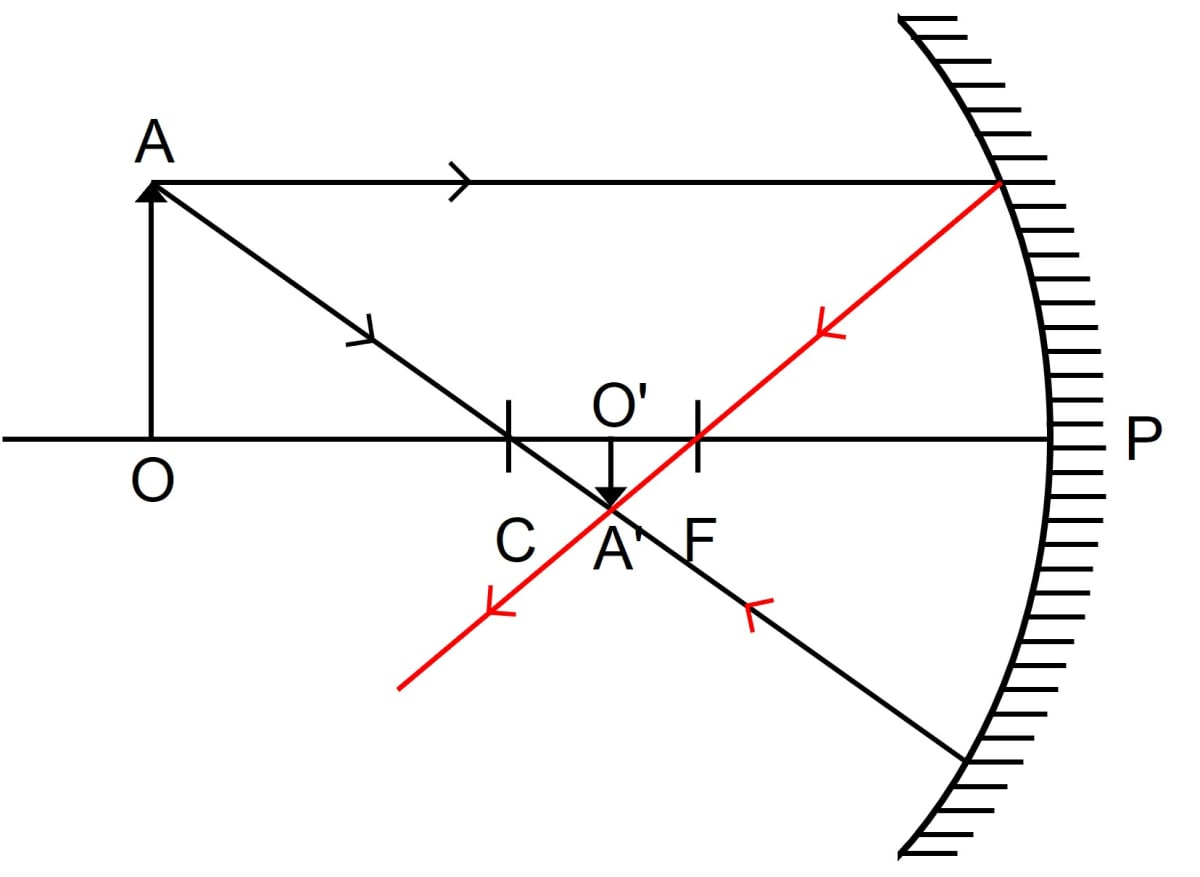#### Question 14

Figure shows a concave mirror with it's pole at P, focus F and center of curvature C. Draw ray diagram to show the formation of image of an object OA.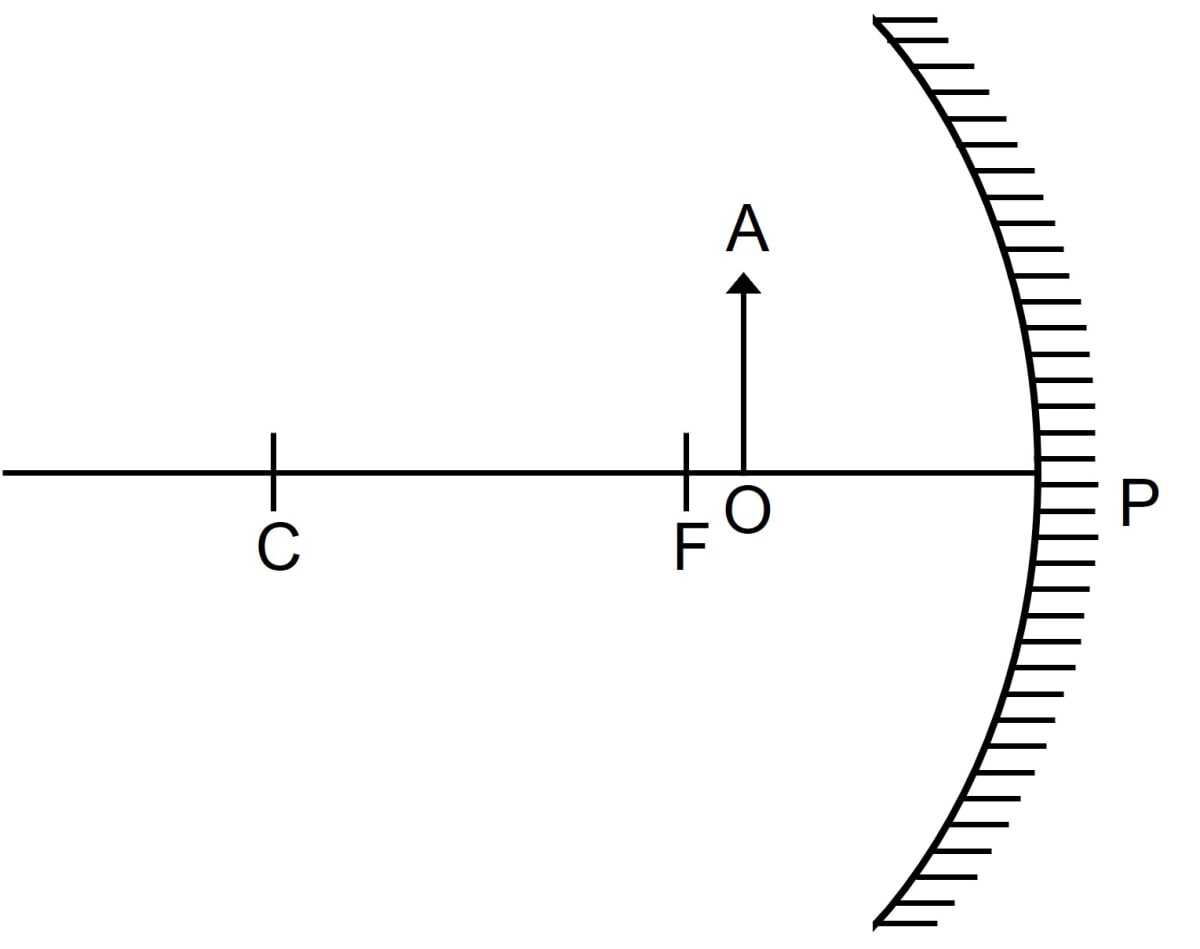Ray diagram showing the formation of the image is given below: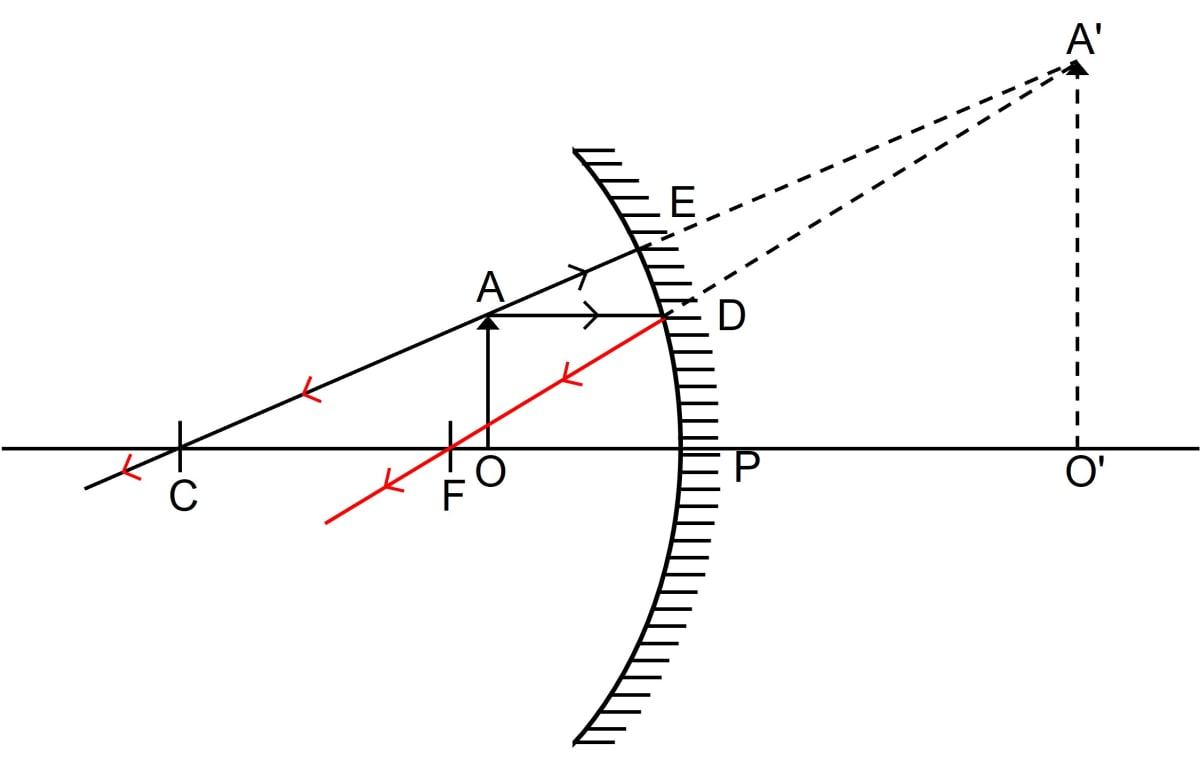#### Question 15

The diagram below in figure shows a convex mirror. C is it's center of curvature and F is it's focus. (i) Draw two rays from A and hence locate the position of image of object OA. Label the image IB. (ii) State three characteristics of the image.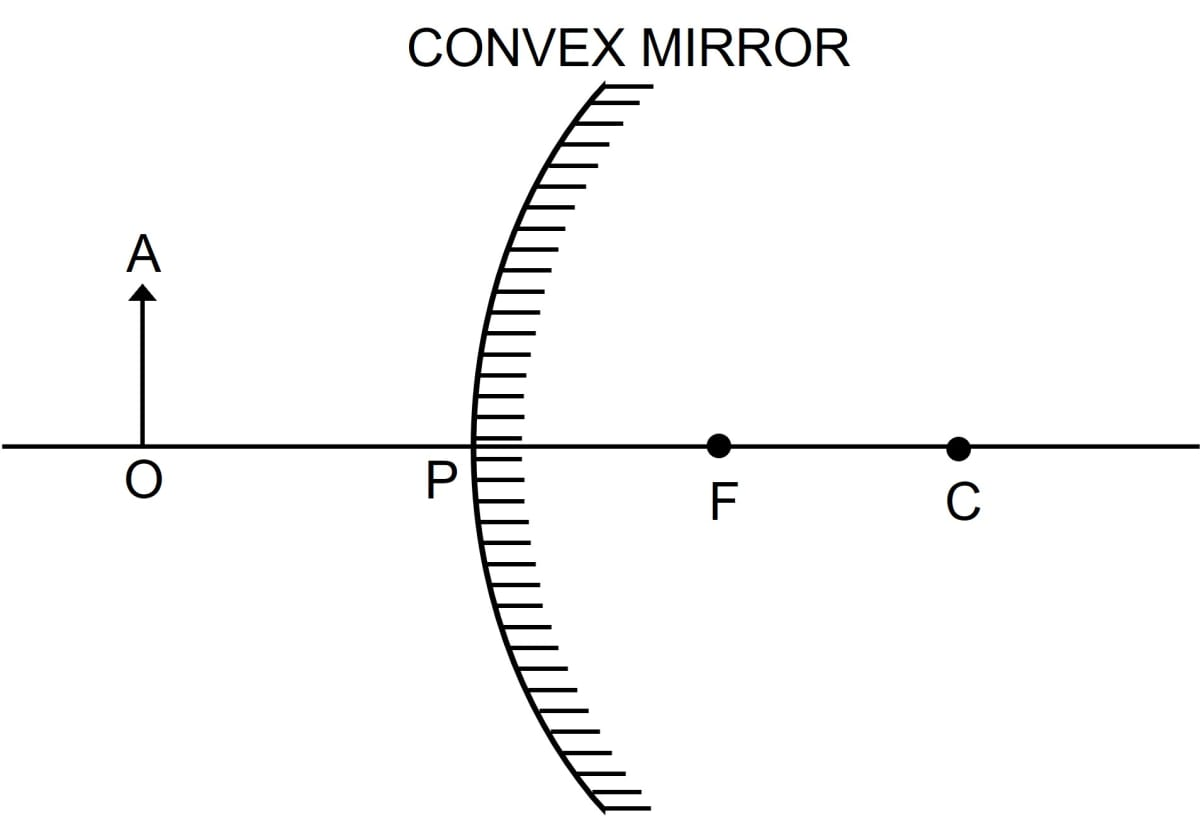(i) Below completed ray diagram shows the position of the image of object OA: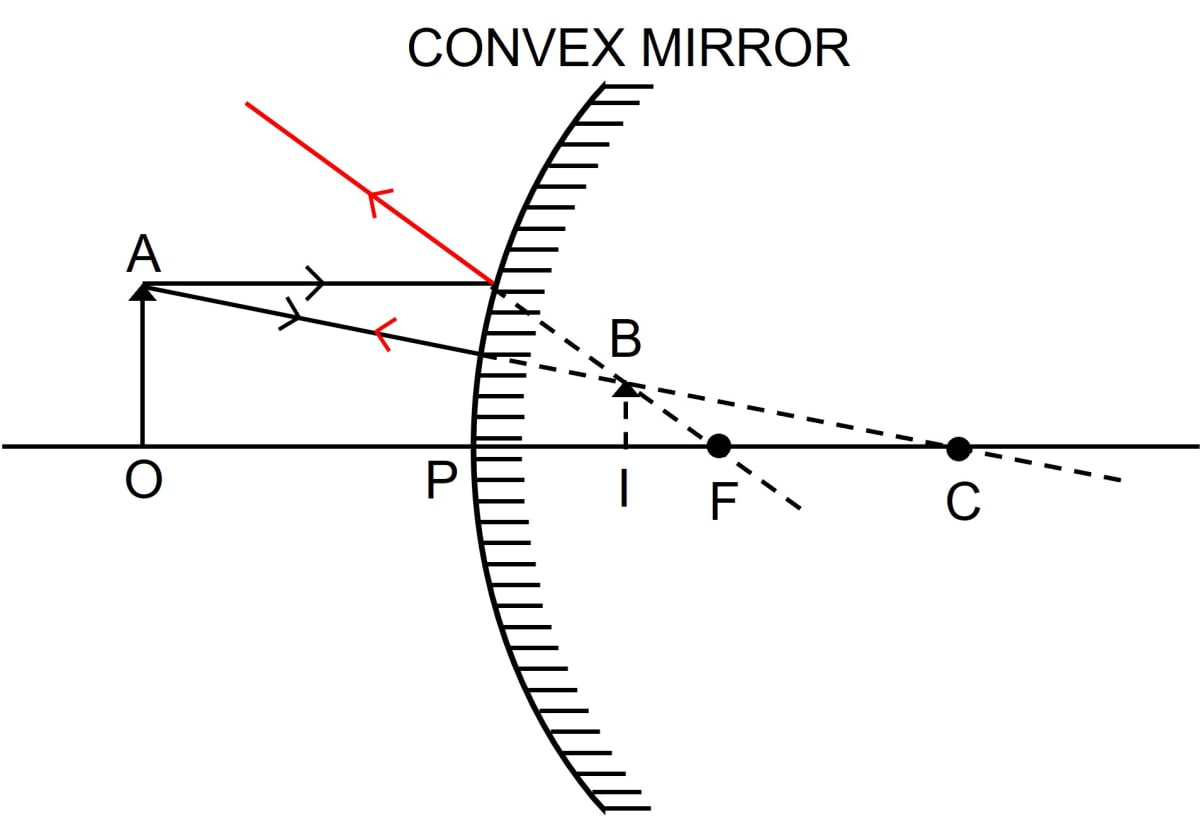(ii) The three characteristics of the image are virtual, upright and diminished.

#### Question 16

Draw a ray diagram to show the formation of image by a concave mirror for an object placed between it's pole and focus. State three characteristics of the image.

Below is the ray diagram showing the formation of image by a concave mirror for an object placed between it's pole and focus:When the object is between the focus F and the pole P, the image is formed behind the mirror. It is virtual, upright and magnified.

#### Question 17

Draw a ray diagram to show the formation of image by a concave mirror for an object beyond it's center of curvature. State three characteristics of the image.

The image by a concave mirror for an object beyond it's center of curvature is shown below:When object is beyond the centre of curvature C, the image is between the focus F and the centre of curvature C. It is real, inverted and diminished.

#### Question 18

Draw a ray diagram to show the formation of image of an object kept in front of a convex mirror. State three characteristics of the image.

The diagram below shows the formation of image when the object is kept in front of a convex mirror.When the object is in in front of the convex mirror, the image is between the pole P and focus F on the other side of the mirror.

The image formed is virtual, upright and diminished.

#### Question 19

Name the mirror which always produces an erect and virtual image. How is the size of image related to the size of object?

A convex mirror always produces an erect and virtual image.

The size of the image is shorter than the size of the object.

#### Question 20

(a) For what position of object, the image formed by a concave mirror is magnified and erect?

(b) State whether the image in part (a) is real or virtual?

(a) When the object is between the pole and focus of a concave mirror then the image formed is magnified and erect

(b) The image is virtual.

#### Question 21

(a) State the position of object for which the image formed by a concave mirror is of same size.

(b) Write two more characteristics of the image.

(a) When the object is at the centre of curvature of a concave mirror, the image is of the same size as the object.

(b) The images thus formed is real and inverted.

#### Question 22

(a) What is a real image?

(b) What type of mirror can be used to obtain a real image of an object?

(c) Does the mirror mentioned in part (b) form real image for all locations of the object?

(a) A real image is one that can be obtained on a screen.

(b) A concave mirror can be used to obtain a real image of an object.

(c) No, concave mirror does not form real image for all the locations of the object.

#### Question 23

Discuss the position and nature of image formed by a concave mirror when an object is moved from infinity towards the pole of mirror.

The image formed moves away from the concave mirror when an object is moved from infinity towards the pole of mirror.

The image is diminished when the object is beyond centre of curvature, but it becomes magnified as the object comes within the centre of curvature. The image is of the same size of the object when the object is at the centre of curvature.

For the object situated beyond focus, the image is always real and inverted, whereas for the object situated between the focus and pole the image is upright and virtual.

The table below shows the position, size and nature of the image formed by a concave mirror for different positions of the object.

No.Position of the objectPosition of the imageSize of the imageNature of the image
1At infinityAt the focusDiminished to a pointReal and inverted
2At very far distanceIn focal planeHighly diminishedReal and inverted
3Beyond the centre of curvatureBetween the centre of curvature and focusDiminishedReal and inverted
4At the centre of curvatureAt the centre of curvatureSame sizeReal and inverted
5Between the centre of curvature and focusBeyond the centre of curvatureMagnifiedReal and inverted
6At focusAt infinityHighly magnifiedReal and inverted
7Between the focus and the poleBehind the mirrorMagnifiedVirtual and upright

#### Question 24

Discuss the position and nature of image formed by a convex mirror when an object is moved from infinity towards the pole of mirror.

In a convex mirror, the image formed is always virtual, upright and diminished. It is always situated between it's pole and focus irrespective of the distance of object in front of the mirror.

As the object comes closer to the mirror from infinity towards the pole, it's image shifts from focus towards the pole and increase in size.

The table below shows the position, size and nature of the image formed by a convex mirror

No.Position of the objectPosition of the imageSize of the imageNature of the image
1.At infinityAt focusDiminished to a pointVirtual and upright
2.At any other pointBetween focus and the poleDiminishedVirtual and upright

#### Question 25

Name the kind of mirror used to obtain —

(a) a real and enlarged image,

(b) a virtual and enlarged image,

(c) a virtual and diminished image,

(d) a real and diminished image.

(a) Concave mirror is used to obtain a real and enlarged image.

(b) Concave mirror is used to obtain a virtual and enlarged image.

(c) Convex mirror is used to obtain a virtual and diminished image.

(d) Concave mirror is used to obtain a real and diminished image.

#### Question 26

How is the focal length of a spherical mirror related to it's radius of curvature?

The focal length 'f' is related to the radius of curvature 'R' in the following way —

$f = \dfrac{1}{2}R$

#### Question 27

Write the spherical mirror's formula and explain the meaning of each symbol used in it.

The formula for the spherical mirror is —

$\dfrac{1}{u} + \dfrac{1}{v} = \dfrac{1}{f}$

u = distance of object

v = distance of image

f = focal length

#### Question 28

What is meant by magnification? Write it's expression. What is it's sign for the (a) real (b) virtual, image?

The ratio of length of the image to the length of object, is called linear magnification.

$\text{Magnification (m)} = \dfrac{\text{Length of image (I)}}{\text{Length of object (O)}} \\[0.5em] = \dfrac{\text{Distance of image (v)}}{\text{Distance of object (u)}} \\[1em] \text{OR} \\[1em] m = \dfrac{I}{O} = - \dfrac{v}{u}$

where

'I' is the length of the image,
'O' is the length of the object,
'v' is the distance of the image,
'u' is the distance of the object.

(a) For a real image, linear magnification m is negative.

(b) For a virtual image, linear magnification m is positive.

#### Question 29

Upto what maximum distance from the pole, the image in a convex mirror can be obtained ? What will be the location of object then ?

The maximum distance from the pole, in a convex mirror where the image can be obtained is till the focal length of the mirror. The object would then has to be at infinity.

#### Question 30

Upto what maximum distance from a concave mirror, the image can be obtained? What will be the location of object for it?

The maximum distance from the concave mirror, where the image can be obtained is infinity. The object would then be at focus.

#### Question 31

How will you distinguish between a plane mirror, a concave mirror and a convex mirror, without touching them?

In order to distinguish between a plane mirror, a concave mirror and a convex mirror, the given mirror is held near the face and the image obtained is seen.

There can be the following three cases —

Case 1 — If image is upright, of same size and it does not change in size by moving the mirror towards or away from the face, then the mirror is plane.

Case 2 — If image is upright, magnified and increases in size on small movement of the mirror away from the face then the mirror is concave.

Case 3 — If image is upright, dimished and decreases in size on small movement of the mirror away from the face then the mirror is convex.

#### Question 32

State two uses of a concave mirror.

Uses of a concave mirror are as follows —

1. As a shaving mirror — When a concave mirror is held near the face (such that the face is between pole and focus of the mirror), it gives an upright and magnified image. Hence even tiny hair can be seen.
2. As a reflector — In torch, searchlight and head light of automobiles, cycles etc., a concave polished metallic surface is used to obtain a parallel beam of light.

#### Question 33

State the kind of mirror used —

(a) by a dentist,

(b) as a search-light reflector.

(a) Concave mirror is used by a dentist.

(b) Concave mirror is used as a search-light reflector.

#### Question 34

(a) When a concave mirror is used as a shaving mirror, where is the person's face in relation to the focus of mirror?

(b) State three characteristics of the image seen in part (a)

(a) When a concave mirror is used as a shaving mirror, the person's face should be between the pole and the focus.

(b) The image formed is erect, virtual and magnified.

#### Question 35

Which mirror will you prefer to use as a rear view mirror in a car — plane mirror or convex mirror? Give one reason.

A convex mirror would be preferred in comparison to a plane mirror for use as a rear view mirror in a car because it provides a much wider field view as compared to a plane mirror of the same size. The below ray diagram shows this: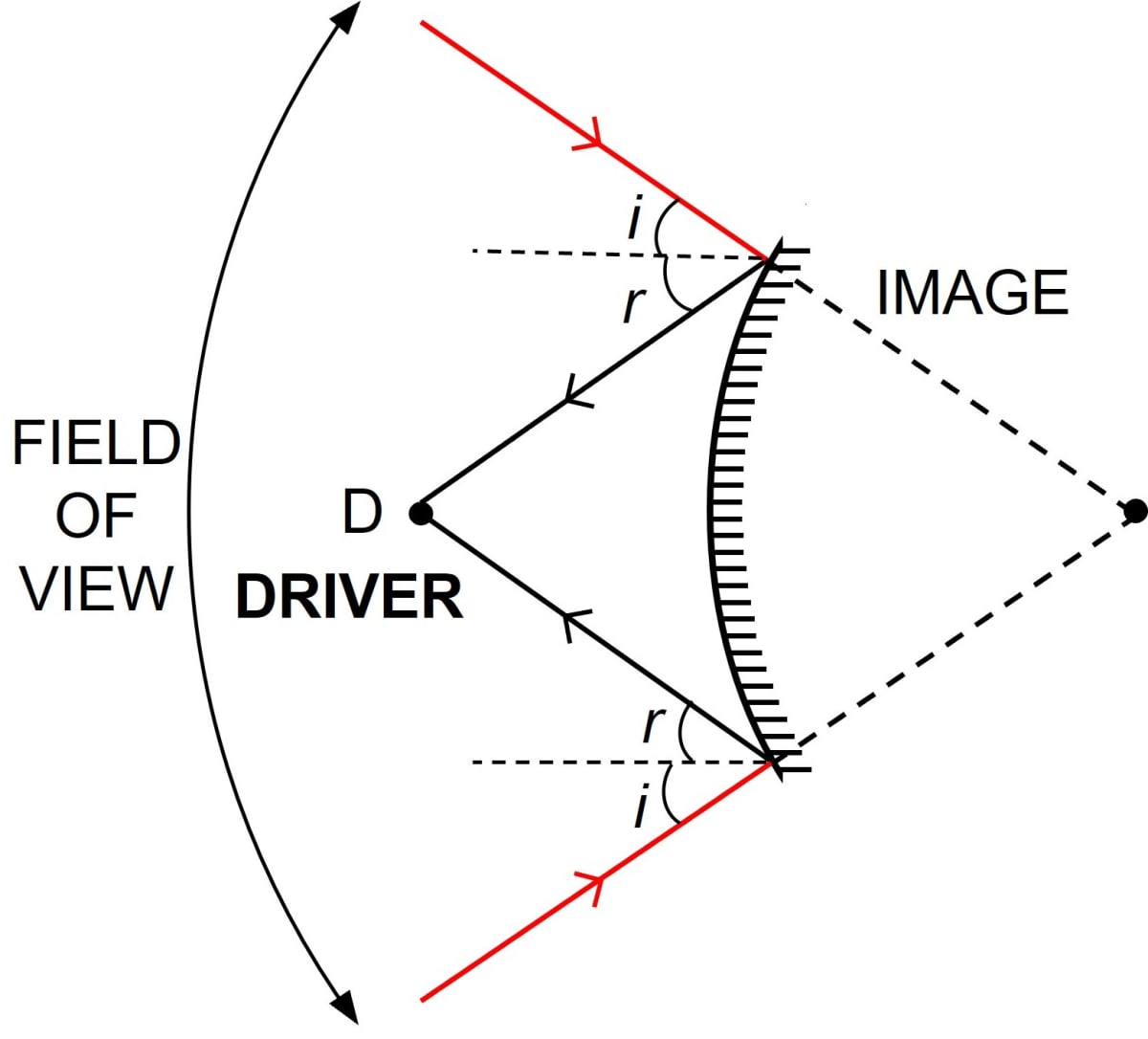#### Question 36

Why does a driver use a convex mirror instead of a plane mirror as a rear view mirror? Illustrate your answer with the help of a ray diagram.

A convex mirror diverges the incident light beam and always forms a virtual, small and erect image behind the mirror between it's pole and focus. This fact enables the driver to use it as a rear view mirror in vehicles to see all the traffic approaching from behind.

Although a plane mirror can also be used as a rear view mirror, but a convex mirror provides a much wider field view as compared to a plane mirror of the same size.

The below diagram shows how a convex mirror provides a better field view than a plane mirror.## Multiple Choice Type

#### Question 1

For an incident ray directed towards center of curvature of a spherical mirror, the reflected ray:

1. retraces it's path ✓
2. passes through the focus
3. passes through the pole
4. becomes parallel to the principal axis

A line joining the centre of curvature to any point on the surface of mirror is normal to the mirror at that point, so a ray AD passing through the center of curvature C (or appearing to pass through through the centre of curvature C) is incident normally on the spherical mirror.
Since it's angle of incidence is zero, therefore the angle of reflection will also be zero and the ray AD gets reflected along it's own path DA as shown below.#### Question 2

The image formed by a convex mirror is:

1. erect and diminished ✓
2. erect and enlarged
3. inverted and diminished
4. inverted and enlarged

In a convex mirror, the image formed is always virtual, erect and diminished. It is always situated between it's pole and focus irrespective of the distance of object in front of the mirror.
As the object comes closer to the mirror from infinity towards the pole, it's image shifts from focus towards the pole and increases in size.

#### Question 3

A real and enlarged image can be obtained by using a:

1. convex mirror
2. plane mirror
3. concave mirror ✓
4. either convex or plane mirror

The image formed by a concave mirror is real and enlarged, when the object is between centre of curvature and focus or at focus.

## Numericals

#### Question 1

The radius of curvature of a convex mirror is 40 cm. find it's focal length.

Focal length = $\dfrac{1}{2}$ x Radius of curvature

Given,

R = 40 cm

Substituting the values in the formula above we get,

$\text{Focal length} = \dfrac{1}{2} \times 40 \\[0.5em] \text{Focal length} = 20 \text{ cm}$

Hence, focal length of the convex mirror = 20 cm.

#### Question 2

The focal length of a concave mirror is 10 cm. Find it's radius of curvature.

Focal length = $\dfrac{1}{2}$ x Radius of curvature

Given,

f = 10 cm

Substituting the values in the formula above we get,

$10 = \dfrac{1}{2} \times \text{radius of curvature} \\[0.5em] \Rightarrow \text{radius of curvature} = 20 \text{ cm}$

Hence, radius of curvature of concave mirror = 20 cm.

#### Question 3

An object of height 2 cm is placed at a distance 20 cm in front of a concave mirror of focal length 12 cm. Find the position, size and nature of the image.

Given,

Object height (O) = 2 cm

Focal length (f) = 12 cm (negative)

Object distance (u) = 20 cm (negative)

Mirror formula:

$\dfrac{1}{u} + \dfrac{1}{v} = \dfrac{1}{f}$

Substituting the values in the formula above we get,

$- \dfrac{1}{20} + \dfrac{1}{v} = - \dfrac{1}{12} \\[0.5em] \dfrac{1}{v} = - \dfrac{1}{12} + \dfrac{1}{20} \\[0.5em] \dfrac{1}{v} = \dfrac{ - 5 + 3 }{60} \\[0.5em] \dfrac{1}{v} = - \dfrac{ 2}{60} \\[0.5em] \dfrac{1}{v} = - \dfrac{1}{30} \\[0.5em] v = - 30 \text{ cm} \\[0.5em]$

Hence, the image is formed at a distance of 30 cm in front of the mirror.

$\text{Magnification (m)} = \dfrac{\text{Length of image (I)}}{\text{Length of object (O)}} \\[0.5em] = \dfrac{\text{Distance of image (v)}}{\text{Distance of object (u)}} \\[1em] \Rightarrow \dfrac{I}{2} = - \dfrac{30}{20} \\[0.5em] \Rightarrow I = -\dfrac{30 \times 2}{20} \\[0.5em] \Rightarrow I = - 3 \text { cm}\\[0.5em]$

Hence, length of image = 3 cm

Image will be real, inverted and magnified.

#### Question 4

An object is placed at 4 cm distance in front of a concave mirror of radius of curvature 24 cm. Find the position of image. Is the image magnified?

Given,

Radius of curvature (R) = 24 cm (negative)

Object distance (u) = 4 cm (negative)

Focal length = $\dfrac{1}{2}$ x Radius of curvature

Substituting the values in the formula above, we get,

$\text{Focal length} = \dfrac{1}{2} \times (- 24) \\[0.5em] \text{Focal length} = - 12 \text{ cm}$

Hence, focal length of the concave mirror = -12 cm.

Mirror formula:

$\dfrac{1}{u} + \dfrac{1}{v} = \dfrac{1}{f}$

Now, substituting the values in the mirror formula , we get,

$- \dfrac{1}{4} + \dfrac{1}{v} = - \dfrac{1}{12} \\[0.5em] \dfrac{1}{v} = - \dfrac{1}{12} + \dfrac{1}{4} \\[0.5em] \dfrac{1}{v} = \dfrac{ - 1 + 3 }{12} \\[0.5em] \dfrac{1}{v} = \dfrac{2}{12} \\[0.5em] \dfrac{1}{v} = \dfrac{1}{6} \\[0.5em] v = 6 \text{ cm} \\[0.5em]$

The image is formed 6 cm behind the mirror.

Computing linear magnification:

$\text{Magnification (m)} = \dfrac{\text{Length of image (I)}}{\text{Length of object (O)}} \\[0.5em] = \dfrac{\text{Distance of image (v)}}{\text{Distance of object (u)}} \\[1em] \Rightarrow m = - \dfrac{6}{- 4} \\[0.5em] \Rightarrow m = \dfrac{6}{4} \\[0.5em] \Rightarrow m = 1.5 \\[0.5em]$

As, the length of image is 1.5 times the image of object, hence, the image is magnified.

#### Question 5

At what distance from a concave mirror of focal length 25 cm should an object be placed so that the size of image is equal to the size of the object.

To get an image of same size the object should be placed at the center of the curvature of a concave mirror.

Given,

focal length = 25 cm

As, centre of curvature = 2 x focal length

Therefore,

centre of curvature

= 2 x 25

= 50 cm

Hence, the object should be kept at 50 cm so that the size of image is equal to the size of object.

#### Question 6

An object 5 cm high is placed at a distance 60 cm in front of a concave mirror of focal length 10 cm. Find (i) the position and (ii) size, of the image.

Given,

Object height (O) = 5 cm

focal length (f) = 10 cm (negative)

Object distance (u) = 60 cm (negative)

Mirror formula:

$\dfrac{1}{u} + \dfrac{1}{v} = \dfrac{1}{f}$

Substituting the values in the formula above, we get,

$- \dfrac{1}{60} + \dfrac{1}{v} = - \dfrac{1}{10} \\[0.5em] \dfrac{1}{v} = - \dfrac{1}{10} + \dfrac{1}{60} \\[0.5em] \dfrac{1}{v} = \dfrac{ - 6 + 1}{60} \\[0.5em] \dfrac{1}{v} = - \dfrac{ 5}{60} \\[0.5em] \dfrac{1}{v} = - \dfrac{1}{12} \\[0.5em] v = - 12 \text{ cm} \\[0.5em]$

The image distance (v) = 12 cm infront of the mirror.

$\text{Magnification (m)} = \dfrac{\text{Length of image (I)}}{\text{Length of object (O)}} \\[0.5em] = \dfrac{\text{Distance of image (v)}}{\text{Distance of object (u)}} \\[1em] \dfrac{I}{5} = - \dfrac{12}{60} \\[0.5em] I = -\dfrac{12 \times 5}{60} \\[0.5em] \Rightarrow I = - 1 \text { cm}\\[0.5em]$

Hence, length of image = 1 cm

Negative sign shows that the image will be inverted.

#### Question 7

A point light source is kept in front of a convex mirror at a distance of 40 cm. The focal length of the mirror is 40 cm. Find the position of image.

Given,

u = 40 cm (negative)

f = 40 cm (positive)

Mirror formula:

$\dfrac{1}{u} + \dfrac{1}{v} = \dfrac{1}{f}$

Substituting the values in the formula above, we get,

$- \dfrac{1}{40} + \dfrac{1}{v} = \dfrac{1}{40} \\[0.5em] \dfrac{1}{v} = \dfrac{1}{40} + \dfrac{1}{40} \\[0.5em] \dfrac{1}{v} = \dfrac{1+1}{40} \\[0.5em] \dfrac{1}{v} = \dfrac{2}{40} \\[0.5em] \dfrac{1}{v} = \dfrac{1}{20} \\[0.5em] \Rightarrow v = 20 \text{ cm} \\[0.5em]$

Hence, the image is formed 20 cm behind the mirror.

#### Question 8

When an object of height 1 cm is kept at a distance 4 cm from a concave mirror, it's erect image of height 1.5 cm is formed at a distance 6 cm behind the mirror. Find the focal length of the mirror.

Given,

u = 4 cm (negative)

v = 6 cm (positive)

Mirror formula:

$\dfrac{1}{u} + \dfrac{1}{v} = \dfrac{1}{f}$

Substituting the values in the formula above, we get,

$- \dfrac{1}{4} + \dfrac{1}{6} = \dfrac{1}{f} \\[0.5em] \dfrac{1}{f} = \dfrac{-3+2}{12} \\[0.5em] \dfrac{1}{f} = - \dfrac{1}{12} \\[0.5em] \Rightarrow f = - 12 \text{ cm} \\[0.5em]$

Hence, the focal length of concave mirror = 12 cm

#### Question 9

An object of length 4 cm is placed in front of a concave mirror at a distance 30 cm. The focal length of mirror is 15 cm. (a) Where will the image form? (b) What will be the length of image?

(a) Given,

u = 30 cm (negative)

f = 15 cm (negative)

Mirror formula:

$\dfrac{1}{u} + \dfrac{1}{v} = \dfrac{1}{f}$

Substituting the values in the formula above, we get,

$- \dfrac{1}{30} + \dfrac{1}{v} = - \dfrac{1}{15} \\[0.5em] \dfrac{1}{v} = - \dfrac{1}{15} + \dfrac{1}{30} \\[0.5em] \dfrac{1}{v} = \dfrac{ - 2 + 1 }{30} \\[0.5em] \dfrac{1}{v} = -\dfrac{1}{30} \\[0.5em] \Rightarrow v = 30 \text{ cm} \\[0.5em]$

Hence, the image is formed at 30 cm in front of the mirror

(b) Magnification

$\text{Magnification (m)} = \dfrac{\text{Length of image (I)}}{\text{Length of object (O)}} \\[0.5em] = \dfrac{\text{Distance of image (v)}}{\text{Distance of object (u)}} \\[1em] \dfrac{I}{4} = - \dfrac{30}{30} \\[0.5em] \dfrac{I}{4} = - 1 \\[0.5em] \Rightarrow I = - 4 \text { cm}\\[0.5em]$

Hence, length of image = 4 cm

Negative sign represents that the image is inverted.

#### Question 10

A concave mirror forms a real image of an object placed in front of it at a distance 30 cm, of size three times the size of object. Find (a) the focal length of mirror (b) position of image.

Given,

(a) Distance of the object (u) = 30 cm (negative)

Image height = 3 times the height of object

So, magnification (m) = 3 (negative for the real image)

$\text{Magnification (m)} = \dfrac{\text{Length of image (I)}}{\text{Length of object (O)}} \\[0.5em] = \dfrac{\text{Distance of image (v)}}{\text{Distance of object (u)}} \\[1em] - 3 = - \dfrac{v}{- 30} \\[0.5em] \Rightarrow v = 3 \times (- 30) \\[0.5em] \Rightarrow v = - 90 \text{ cm} \\[0.5em]$

Hence, the image is formed 90 cm in front of the mirror.

Mirror formula:

$\dfrac{1}{u} + \dfrac{1}{v} = \dfrac{1}{f}$

Substituting the values in the mirror formula we get,

$- \dfrac{1}{30} - \dfrac{1}{90} = \dfrac{1}{f} \\[0.5em] \dfrac{1}{f} = \dfrac{ - 3 - 1 }{90} \\[0.5em] \dfrac{1}{f} = -\dfrac{4}{90} \\[0.5em] \Rightarrow f = -22.5 \text{ cm} \\[0.5em]$

Hence, focal length of the mirror = 22.5 cm

(b) The image is formed 90 cm in front of the mirror

#### Question 11

A concave mirror forms a virtual image of size twice that of the object placed at a distance 5 cm from it. Find (a) the focal length of the mirror (b) position of the image.

(a) Given,

Distance of the object (u) = 5 cm (negative)

Magnification (m) = 2 (positive for the virtual image)

$\text{Magnification (m)} = \dfrac{\text{Length of image (I)}}{\text{Length of object (O)}} \\[0.5em] = \dfrac{\text{Distance of image (v)}}{\text{Distance of object (u)}} \\[1em] 2 = - \dfrac{v}{- 5} \\[0.5em] \Rightarrow v = 2 \times 5 \\[0.5em] \Rightarrow v = 10 \text{ cm} \\[0.5em]$

Hence, the image is formed 10 cm behind the mirror.

Mirror formula:

$\dfrac{1}{u} + \dfrac{1}{v} = \dfrac{1}{f}$

Substituting the values in the mirror formula, we get,

$- \dfrac{1}{5} + \dfrac{1}{10} = - \dfrac{1}{f} \\[0.5em] - \dfrac{1}{f} = \dfrac{ - 2 + 1 }{10} \\[0.5em] - \dfrac{1}{f} = -\dfrac{1}{10} \\[0.5em] \Rightarrow f = 10 \text{ cm} \\[0.5em]$

Hence, focal length of the mirror = 10 cm

(b) The position of the image is 10 cm behind the mirror

#### Question 12

The image formed by a convex mirror is of size one-third the size of object. How are u and v related?

Magnification (m) = - $\dfrac{\text {image distance (v)}}{\text{object distance (u)}}$ = - $\dfrac{1}{3}$

Hence, we get,

v = - $\dfrac{1}{3}$u

Or

u = -3v

#### Question 13

The erect image formed by a concave mirror is of size double the size of object. How are u and v related?

Magnification (m) = - $\dfrac{\text {image distance (v)}}{\text{object distance (u)}}$

Given,

2 = - $\dfrac{v}{u}$

Hence, v = -2u

#### Question 14

The magnification for a mirror is -3. How are u and v related?

Magnification (m) = - $\dfrac{\text {image distance (v)}}{\text{object distance (u)}}$
$-3 = -\dfrac{\text{v}}{\text{u}} \\[0.5em] \Rightarrow \text{v} = \text{3u}$